# Assessment of an isogeometric approach with Catmull–Clark subdivision surfaces using the Laplace–Beltrami problems

## Abstract

An isogeometric approach for solving the Laplace–Beltrami equation on a two-dimensional manifold embedded in three-dimensional space using a Galerkin method based on Catmull–Clark subdivision surfaces is presented and assessed. The scalar-valued Laplace–Beltrami equation requires only $$C^0$$ continuity and is adopted to elucidate key features and properties of the isogeometric method using Catmull–Clark subdivision surfaces. Catmull–Clark subdivision bases are used to discretise both the geometry and the physical field. A fitting method generates control meshes to approximate any given geometry with Catmull–Clark subdivision surfaces. The performance of the Catmull–Clark subdivision method is compared to the conventional finite element method. Subdivision surfaces without extraordinary vertices show the optimal convergence rate. However, extraordinary vertices introduce error, which decreases the convergence rate. A comparative study shows the effect of the number and valences of the extraordinary vertices on accuracy and convergence. An adaptive quadrature scheme is shown to reduce the error.

## Introduction

Hughes et al.  proposed the concept of isogeometric analysis (IGA) in 2005. The early works on IGA [10, 18, 47] focussed on geometries modelled using Non-Uniform Rational B-Splines (NURBS) as these are widely used in computer aided design (CAD). NURBS can be used to model free-form, two-dimensional curves. However, a NURBS surface is a tensor product surface generated by two NURBS curves, thereby imposing limitations for modelling complex geometries with arbitrary topologies. Complex CAD models are always composed of a number of NURBS patches. These patches are often poorly connected in the design stage. When such models are used for analysis, the unmatched patches must be treated carefully to ensure the geometries are watertight. Furthermore, because NURBS can not be locally refined, adaptive mesh refinement method cannot be employed. A number of alternative CAD techniques were developed and adopted in IGA to overcome these limitations, including Hierarchical B-splines [29, 52], T-splines [11, 49], PHT-splines [23, 42], THB-splines [13, 30] and LR B-splines [24, 34]. Some of these recent techniques are being adopted by the engineering design market. However, the majority are the subject of academic research and not widely used in the CAD community. Moreover, computing the basis functions for analysis using these alternative approaches can be expensive. Catmull and Clark  developed a bicubic B-spline patch subdivision algorithm for describing smooth three dimensional objects. The use of Catmull–Clark subdivision surfaces to model complex geometries in the animation and gaming industries dates back to 1978. Catmull–Clark subdivision surfaces can be considered as uniform bi-cubic splines which can be efficiently evaluated using polynomials.

In CAD, distortion of regular parametrizations are inevitable and indeed vital when modelling complex geometries. Allowing ‘extraordinary vertices’ ensures that Catmull–Clark subdivision surfaces can be used for modelling complex geometries with arbitrary topology. Cirak et al.   implemented Loop subdivision surfaces for solving the Kirchhoff–Love shell formulation. This was the first application of subdivision surfaces to engineering problems. Subdivision surfaces have subsequently been used in electromagnetics , shape optimisation [6, 7] , acoustics [15, 38] and lattice-skin structures .

Catmull–Clark subdivision surfaces face a number of challenges when used for analysis. Many of these have been discussed in the literature, however a unified assessment is lacking. This manuscript provides a clear and concise discussion of the challenges and limitations of Catmull–Clark subdivision surfaces.

Engineering designs often require exact geometries including circles, spheres, tori and cones. However, subdivision surfaces can not capture these geometries exactly. Moreover, there are always offsets between the control meshes and the surfaces. Fitting subdivision surfaces  aim to overcome this limitation. Although the fitting subdivision surfaces still can not model arbitrary geometries exactly as they are interpolated using cubic splines, they can approximate the given geometries closely through least-square fitting. Another challenge of subdivision surfaces is that they can model smooth closed manifolds easily but require special treatment to model manifolds with boundaries. A common solution is to introduce ‘ghost’ control vertices to provide bases for interpolating. From the perspective of analysis, the shape functions will span into ‘ghost’ elements . In addition, the spline basis functions do not possess an interpolating property. Thus it is difficult to directly impose Dirichlet boundary conditions. Meshless methods and extended finite element methods have developed strategies to overcome this problem [28, 39]. A common strategy is to modify the weak form of the governing equation. Methods include the Lagrangian multiplier method , the penalty method  and Nitsche’s method [32, 43].

Conventional Catmull–Clark subdivision surfaces can not be locally refined. Truncated hierarchical Catmull–Clark subdivision surfaces (THCCS), developed by Wei et al.  , overcome this limitation. They generalise truncated hierarchical B-splines (THB-splines) to meshes with arbitrary topology. Wei et al.   subsequently improved their method using a new basis function insertion scheme and thereby enhanced the efficiency of local refinement. The extraordinary vertices introduce singularities in the parametrisation [41, 51]. Catmull–Clark subdivision surfaces have $$C^2$$ continuity everywhere except at the surface points related to extraordinary vertices where, as demonstrated by Peters and Reif  , they possess $$C^1$$ continuity. Stam   developed a method to evaluate Catmull–Clark subdivision surfaces directly without explicitly subdividing, thus allowing one to evaluate elements containing extraordinary vertices. Although the surface gradients can not be evaluated at the extraordinary vertices, they can be evaluated at nearby quadrature points. Thus, subdivision surfaces can be used as $$C^1$$ elements as required, for example, in thin shell theory . Nevertheless, the evaluation of points around extraordinary vertices of Catmull–Clark surfaces introduces error. The conventional evaluation method repeatedly subdivides the element patch until the target point fall into a regular patch allowing a uniform bi-cubic B-spline patch to be mapped the subdivided element patch. The extraordinary vertex also introduces approximation errors because of the singular parameterisations at extraordinary vertices [40, 44]. Stam’s natural parametrisation only can achieve $$C^0$$ continuity at extraordinary vertices. Recently Wawrzinek and Polthier   introduced a characteristic subdivision finite element scheme that adopted a characteristic reparameterisation for elements with extraordinary vertices. The evaluated limiting surface is at least $$C^1$$ everywhere and the numerical accuracy is improved. Zhang et al.   optimised the subdivision scheme to improve its approximation properties when used for thin-shell theory.

Using the finite element method to solve the partial differential equations (PDEs) on surfaces dates back to the seminal work by Dziuk  , which developed a variational formulation to approximate the solution of the Laplace–Beltrami problems on two dimensional surfaces. This method was extended to solve nonlinear and higher-order equations on surfaces by Dziuk and Elliott  . Dziuk and Elliott   also provided a thorough review on finite element methods for approximating the solution of PDEs on surfaces. Dedner et al.   proposed a discontinuous Galerkin (DG) method for solving a elliptic problem with the Laplace–Beltrami operator on surfaces. Adaptive DG  and high-order DG  methods were also developed for solving PDEs on surfaces. However, the accuracy of these methods depends on the approximation of the mean curvatures of the surfaces. The geometrical error is dominant when conventional Lagrangian discretisation is used to approximate solutions on complex surfaces. Isogeometric discretisation maintains the exact geometry and overcomes this limitation. Dedè and Quarteroni   proposed an isogeometric approach for approximating several surface PDEs involving the Laplace–Beltrami operator on NURBS surfaces. Bartezzaghi et al.   solved PDEs with high order Laplace–Beltrami operators on surfaces using NURBS based isogeometric Galerkin method. More accurate results are obtained using an IGA approach over the conventional finite element method. Langer et al.   present an isogeometric DG method with non-matching NURBS patches allowing the approximation of PDEs on more complex surfaces.

This work presents a thorough and unified discussion of several major issues related to isogeometric Galerkin formulation based on Catmull–Clark subdivision surfaces. The difficulties associated with imposing Dirichlet boundary conditions, the reduction of the approximation power around extraordinary vertices, and the problem of sufficient numerical integration in the element with extraordinary vertices will be examined and discussed. Previous studies [16, 17] on Catmull–Clark subdivision surfaces for analysis introduce ghost degrees of freedoms for constructing basis functions in elements at boundaries. We propose a method which modifies the basis functions at boundaries to ensure they are only associated with given control vertices. No additional ghost degrees of freedom are involved. A penalty method is employed to impose Dirichlet boundary conditions. This does not change the size or symmetry of the system matrix and is straightforward to implement. An adaptive quadrature scheme inspired by  is presented to increase the integration accuracy for elements with extraordinary vertices. The proposed method can perform isogeometric analysis on complex geometries using Catmull–Clark subdivision discretisations. A test for approximating Poisson’s problem on a square plate is conducted to demonstrate the properties of the method in a simplified setting so as to distill the key features. The approach is also used for solving the Laplace–Beltrami equation which is a benchmark problem for curved manifolds [35, 41]. A comparative convergence study is conducted between the Catmull–Clark subdivision method and the conventional finite element method. The effects of the extraordinary vertices and modified bases at boundaries on convergence are examined. Catmull–Clark subdivision surfaces are limiting surfaces generated by successively subdividing given control meshes. They are identical to uniform bi-cubic B-splines. Thus, they have difficulty to represent desired geometries exactly. Here, a least-squares fitting method is used to fit any given geometry using Catmull–Clark subdivision surfaces.

This manuscript first summarises the subdivision algorithm and the evaluation method for Catmull–Clark subdivision surfaces. Then, techniques for using Catmull–Clark for numerical analysis and improving accuracy are presented in Sect. 3. Section 4 presents the Laplace–Beltrami problem and Sect. 5 shows a Galerkin method with Catmull–Clark subdivision surface bases. Section 6 showcases the numerical results.

## Catmull–Clark subdivision surfaces

There exist a variety of subdivision schemes, but the basic idea is to use a subdivision scheme to generate a smooth surface through a limiting procedure of repeated refinement steps starting from an initial polygonal grid. The Catmull–Clark algorithm can generate curves and surfaces which are identical to cubic B-splines. The algorithms for curves and surfaces are shown in Appendices A.1 and A.2, respectively. This section will briefly introduce the methods for interpolating and evaluating curves and surfaces using the Catmull–Clark subdivision algorithm.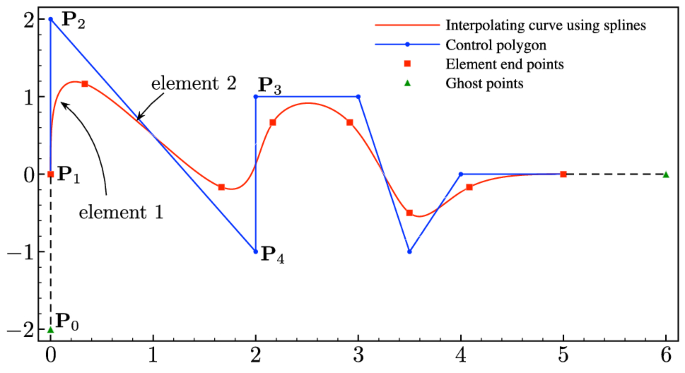Fig. 1

### Curve interpolation and evaluation based on the subdivision algorithm

Figure 1 shows a curve generated using a subdivision algorithm. The interpolated curve is identical to a cubic B-spline curve. The limiting curve can be interpolated using cubic basis splines and associated control points. With a control polygon containing n control points, the curve is naturally divided into $$n-1$$ elements. Each element in the curve is associated with one segment of the control polygon. To interpolate on the target element, four control points including the neighbouring control points are required. For example, if one aims to evaluate the geometry of element 2 in Fig. 1, the four control points $${\mathbf {P}}_1$$,$${\mathbf {P}}_2$$,$${\mathbf {P}}_3$$ and $${\mathbf {P}}_4$$ are required and the curve point is evaluated as

\begin{aligned} {\mathbf {x}}(\xi ) = \sum _{A = 1}^4 N_A(\xi ) {\mathbf {P}}_A, \end{aligned}
(1)

where $$\xi \in [0,1]$$ is the parametric coordinate within an element. The basis functions for element 2 are defined by

\begin{aligned} N_1(\xi )&= \frac{1}{6}[1-3\xi +3\xi ^2-\xi ^3], \nonumber \\ N_2(\xi )&= \frac{1}{6}[4-6\xi ^2+3\xi ^3], \nonumber \\ N_3(\xi )&= \frac{1}{6}[1+3\xi +3\xi ^2-3\xi ^3], \quad N_4(\xi ) = \frac{1}{6}\xi ^3. \end{aligned}
(2)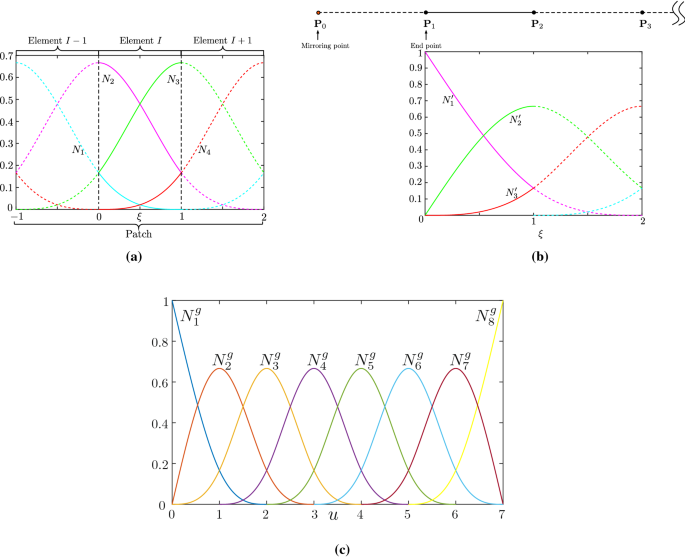Fig. 2

The bases are visualised in Fig. 2a. They are $$C^2$$ continuous across element boundaries. Element 1 in Fig. 1 contains the end of the curve, which has an end curve point that coincides with the control point. In order to evaluate this curve, one needs to mirror the point $${\mathbf {P}}_2$$ to $${\mathbf {P}}_0$$ as

\begin{aligned} {\mathbf {P}}_0 = 2{\mathbf {P}}_1 - {\mathbf {P}}_2. \end{aligned}
(3)

The curve point can now be evaluated using basis splines with a set of control points shown in Fig. 2b. However, if one adopts a spline discretisation for analysis, this strategy of end element treatment will introduce additional ‘ghost-like’ degrees of freedom. To avoid this problem, the expression for $${\mathbf {P}}_0$$ (3) is substituted into the interpolating equation yielding

\begin{aligned} {\mathbf {x}}(\xi ) = \sum _{A=0}^3 N_{A+1}(\xi ) {\mathbf {P}}_A = \sum _{B = 1 }^3 N'_B(\xi ){\mathbf {P}}_B. \end{aligned}
(4)

Hence only three control points are required to evaluate a curve point and the modified basis functions for interpolating end elements are defined by

\begin{aligned} N'_1(\xi )&= \frac{1}{6}[6-6\xi +\xi ^3], \quad N'_2(\xi ) = \frac{1}{6}[6\xi - 2\xi ^3], \nonumber \\ N'_3(\xi )&= \frac{1}{6}\xi ^3. \end{aligned}
(5)

Figure 2b illustrates the modified basis functions. It achieves the same basis functions as the cubic B-Spline with $$p+1$$ multiple knots at the two end points. The new basis functions do not possess the Kronecker delta property but do have the interpolating property at the boundary. The performance of modified bases in analysis will be discussed in Sect. 6.1.Fig. 3

The global basis functions for interpolating the curve in Fig. 1 are shown in Fig. 2c. It is worth noting that this subdivision curve is a cubic B-spline curve and represents a special case of Lane–Riesenfeld subdivision it can not model conical shapes exactly. This property is significantly different to NURBS and motivates Sect. 3.1 on geometry fitting.

### Interpolating and evaluating Catmull–Clark subdivision surfaces

One defines the number of elements connected with the vertex as the valence. A regular vertex in a Catmull–Clark surface mesh has a valence of 4. A vertex with a valence not equal to 4 is called an extraordinary vertex. This allows subdivision surfaces to handle arbitrary topologies. In their seminal paper , Catmull and Clark proposed a way to modify the weight distributions for extraordinary vertices in order to describe complex geometries. With this simple solution, Catmull–Clark surfaces can use a single mesh to present surfaces of arbitrary geometries while other spline-based CAD tools, such as NURBS surfaces, need to link multiple patches. The limiting surface of the Catmull–Clark subdivision algorithm has $$C^2$$ continuity over the surface except at the extraordinary vertices where they have $$C^1$$ continuity as proven by Peters and Reif  . This section will illustrate the methods of interpolating and evaluating both regular element and element with an extraordinary vertex in Catmull–Clark subdivision surfaces.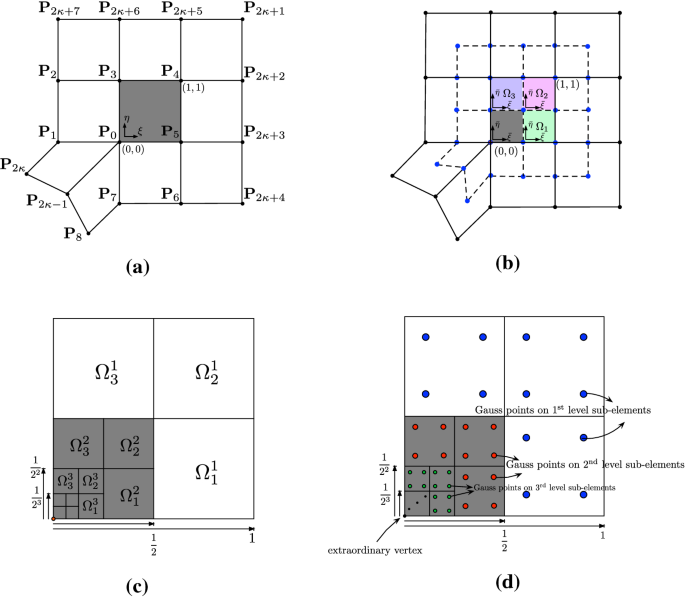Fig. 4

#### Element in a regular patch

Figure 3a shows a subdivision surface element (dashed) which does not contain an extraordinary vertex. In order to evaluate a point in this Catmull–Clark element, an element patch must be formed. The patch consists of the element itself and the elements which share vertices with it. A regular element patch has 9 elements with 16 control vertices. The surface point can be evaluated using the 16 basis functions associated with these control points as

\begin{aligned} {\mathbf {x}}(\varvec{\xi }) = \sum _{A = 0}^{15} N_A(\varvec{\xi }) {\mathbf {P}}_A, \end{aligned}
(6)

where $$\varvec{\xi }:=(\xi ,\eta )$$ is the parametric coordinate of a Catmull–Clark subdivision surface element. A Catmull–Clark surface is obtained as the tensor product of two Catmull–Clark curves. The basis functions are defined by

\begin{aligned} N_i(\varvec{\xi }) = N_{i\%4}(\xi )N_{\lfloor i/4 \rfloor }(\eta ), \, i =0,1,\ldots ,15, \end{aligned}
(7)

where $$N(\xi )$$ or $$N(\eta )$$ are the basis functions defined in Eq. (2) and presented in Fig. 3a. $$\lfloor \bullet \rfloor$$ is the modulus operator and % denotes the remainder operator which gives the remainder of the integer division.

Figure 3b shows the element patch of a subdivision surface element (shaded) that has an edge on the physical boundary. This type of element has only 5 neighbour elements so that it belongs to an element patch which has 12 control vertices. To evaluate this element, a common solution is to generate a set of ‘ghost’ vertices outside the domain to form a full element patch . However, this method involves additional degrees of freedom in numerical analysis. Instead, the curve basis functions in Eq. (5) are adapted to deal with the element on the boundary. The same strategy is used for elements which have two edges on the physical boundary as shown in Fig. 3c.

#### Element in a patch with an extraordinary vertex

Extraordinary vertices are a key advantage of Catmull–Clark subdivision surfaces which allows them to model complex geometries with arbitrary topologies. However, it increases the difficulty of evaluating the surfaces. Figure 4a shows a Catmull–Clark subdivision element which contains one extraordinary vertex.

In order to evaluate this element, one needs to re-numerate the control points as shown in Fig. 4a. After applying one level of subdivision, new control points are generated and this element is subdivided into four sub-elements, as shown in Fig. 4b. The sub-elements $${\varOmega }_1$$, $${\varOmega }_2$$ and $${\varOmega }_3$$ are now in a regular patch. However, the last sub-element (grey) still has an extraordinary vertex. If the target point to be evaluated is in this region, we must repeatedly subdivide the element until the point falls into a sub-element with a regular patch. Then, the point can be evaluated within the sub-element with the new set of control points $${\mathscr {P}}_{n,k}$$, where n is the number of subdivision required and $$k = 1, 2, 3$$ is the sub-element index shown in Fig. 4b. The new control point set is computed as

\begin{aligned} {\mathscr {P}}_{n,k} = {{{{\mathbf {\mathsf{{D}}}}}}_k {{{\mathbf {\mathsf{{A}}}}}} \bar{{{{\mathbf {\mathsf{{A}}}}}}}^{n-1}} {\mathscr {P}}_0, \end{aligned}
(8)

where $${{{\mathbf {\mathsf{{D}}}}}}_k$$ is a selection operator to pick control points for the sub-elements. $${{{\mathbf {\mathsf{{A}}}}}}$$ and $$\bar{{{{\mathbf {\mathsf{{A}}}}}}}$$ are two types of subdivision operators. $${\mathscr {P}}_0$$ is the initial set of control points. The detailed approach is given in  and also can be found in Appendix A.3. $${\mathscr {P}}_{n,k}$$ contains 16 control points. Then, a surface point in the element with an extraordinary vertex can be computed as

\begin{aligned} {\mathbf {x}}(\varvec{\xi }) = \sum _{A=0}^{15} N_A(\bar{\varvec{\xi }})\, {\mathbf {P}}^{n,k}_{A}, \end{aligned}
(9)

where $$\bar{ \varvec{ \xi }}$$ is the parametric coordinates of the evaluated point in the sub-element, which can be mapped from $$\varvec{ \xi }$$ as

\begin{aligned} \bar{ \varvec{ \xi }} = \left\{ \begin{array}{ll} (2^n\xi - 1, 2^n\eta ) &{}\quad \text {if} \quad k =1 \\ (2^n\xi - 1, 2^n\eta -1) &{}\quad \text {if} \quad k =2\\ (2^n\xi , 2^n\eta -1) &{}\quad \text {if} \quad k =3 \end{array} \right. . \end{aligned}
(10)

Equation (9) can thus be rewritten as

\begin{aligned} {\mathbf {x}}(\varvec{\xi }) = \sum _{A=0}^{2\kappa +7} {\hat{N}}_A( {\varvec{ \xi }})\, {\mathbf {P}}^{0}_{A}, \end{aligned}
(11)

where $${\hat{N}}$$ is the Catmull–Clark subdivision surfaces basis function. Define $$\hat{{\mathbf {N}}}$$ as a set of $$2\kappa +8$$ basis functions in an element with an extraordinary vertex and $${\mathbf {N}}$$ is a set of 16 regular basis functions defined in Eq. (7). $$\hat{{\mathbf {N}}}$$ can be calculated in a vector form as

\begin{aligned} \hat{{\mathbf {N}}}(\varvec{ \xi }) = {[{{{\mathbf {\mathsf{{D}}}}}}_k {{{\mathbf {\mathsf{{A}}}}}} \bar{{{{\mathbf {\mathsf{{A}}}}}}}^{n-1}]^T {\mathbf {N}}}(\bar{ \varvec{ \xi }}). \end{aligned}
(12)

The derivatives of the Catmull–Clark subdivision surfaces basis functions for elements containing extraordinary vertices are expressed as

\begin{aligned} \frac{\partial \hat{{\mathbf {N}}}(\varvec{ \xi }) }{\partial \mathbf {\varvec{\xi }}}=\left[ \begin{array}{cc} \frac{\partial {\hat{N}}_0}{\partial \xi } &{} \frac{\partial {\hat{N}}_0}{\partial \eta } \\ \frac{\partial {\hat{N}}_1}{\partial \xi } &{} \frac{\partial {\hat{N}}_1}{\partial \eta } \\ \vdots &{} \vdots \\ \frac{\partial {\hat{N}}_{2\kappa +7}}{\partial \xi } &{} \frac{\partial {\hat{N}}_{2\kappa +7}}{\partial \eta } \\ \end{array} \right] , \end{aligned}
(13)

and can be computed by

\begin{aligned} \frac{\partial \hat{{\mathbf {N}}}(\varvec{ \xi }) }{\partial \mathbf {\varvec{\xi }}} =[{{{\mathbf {\mathsf{{D}}}}}}_k {{{\mathbf {\mathsf{{A}}}}}} \bar{{{{\mathbf {\mathsf{{A}}}}}}}^{n-1}]^T \frac{\partial {{{\mathbf {N}}}}(\bar{\varvec{ \xi }})}{\partial \bar{ \varvec{ \xi }}} \frac{\partial \bar{\varvec{\xi }}}{\partial \varvec{\xi }}, \end{aligned}
(14)

where $$\frac{\partial \bar{\varvec{\xi }}}{\partial \varvec{\xi }}$$ can be considered as a mapping matrix defined by

\begin{aligned} \frac{\partial \bar{\varvec{\xi }}}{\partial \varvec{\xi }} = \left[ \begin{array}{cc} 2^n &{} 0\\ 0 &{} 2^n \end{array} \right] . \end{aligned}
(15)

### Remark 1

The calculation of the basis functions $$\hat{{\mathbf {N}}}$$ at a physical point $${\mathbf {x}}$$ involves two mappings. The first is from the physical domain to the parametric domain of an element with an irregular patch, $${\mathbf {x}} \mapsto \varvec{\xi }$$. Because the irregular patch does not have the tensor-product nature, n levels of subdivisions are required and the point is mapped to the parametric domain of a sub-element, $$\varvec{\xi } \mapsto \bar{\varvec{\xi }}$$. This second mapping is defined in Eq. (10). The value of n approaches positive infinity when $$\varvec{\xi }$$ approaches the extraordinary vertex which has the parametric coordinate (0, 0). Hence the diagonal terms in the mapping matrix (15) tend to positive infinity as $$n \rightarrow \infty$$. This results in the basis functions $$\hat{{\mathbf {N}}}$$ not being differentiable at $$\varvec{\xi } = {\mathbf {0}}$$. This problem is termed singular configuration in , and singular parameterisation in [41, 51].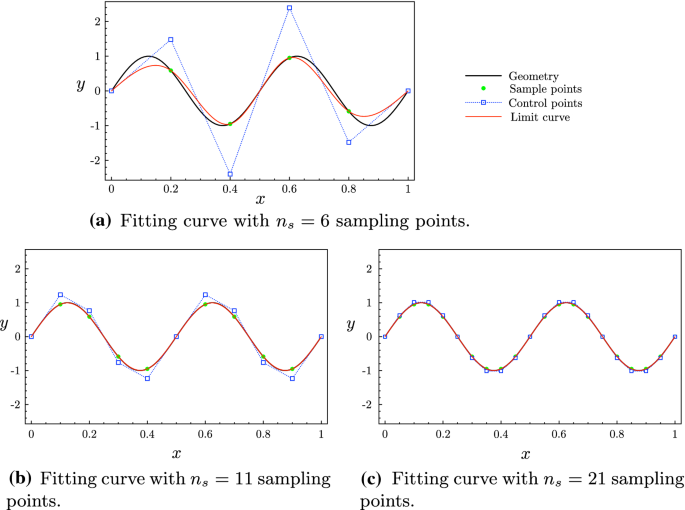Fig. 5

## Techniques for analysis and improving accuracy

This section presents three techniques which are essential for using Catmull–Clark subdivision surfaces in numerical analysis. A geometry fitting method using Catmull–Clark surfaces is introduced in Sect. 3.1. Section 3.2 illustrates an adaptive quadrature scheme for integrating element with an extraordinary vertex to improve accuracy. Section 3.3 introduces the penalty method for applying essential boundary conditions.

### Geometry fitting

Catmull–Clark subdivision surfaces are CAD tools which can construct limiting surfaces from control polygons and meshes. However, in a number of engineering problems, the geometry is given as an industry design and a limit surface that is a “best approximation” of this desired geometry required. Litke et al.   introduced a method for fitting a Catmull–Clark subdivision surface to a given shape. They employed, both a least-squares fitting method and a quasi-interpolation method to determine a set of control points for a given surface. The least-square fitting method is used here. One first chooses a set of sample points $${\mathscr {S}} = \{ {\mathbf {s}}_1, {\mathbf {s}}_2, \ldots , {\mathbf {s}}_{n_s} \} \in {\varGamma }$$, where $${\varGamma }$$ is the geometry, $$n_s$$ is the number of sample points. Each sample point should be evaluated using Catmull–Clark subdivision bases with control points as

\begin{aligned} {\mathbf {s}}(\varvec{ \xi }) =\sum _{A = 1}^{n_b} N_{A}(\varvec{ \xi }){\mathbf {P}}_{A}, \end{aligned}
(16)

where $$n_b = 2\kappa +8$$ is the number of local basis functions. Then the set of sample points can be evaluated as

\begin{aligned} {\mathscr {S}} = {{\mathbf {\mathsf{{L}}}}} {\mathscr {P}}, \end{aligned}
(17)

where $${\mathscr {P}} = \{ {\mathbf {P}}_1, {\mathbf {P}}_2, \ldots , {\mathbf {P}}_{n_c} \}$$ is a set of control points with $$n_c$$ control points. $${{{\mathbf {\mathsf{{L}}}}}}$$ is an evaluation operator of Catmull–Clark curves or surfaces. Set $$\varvec{ \xi } = (0,0)$$ to ensure the sample points correspond to the control vertices and $$n_s \equiv n_c$$, then $${{{\mathbf {\mathsf{{L}}}}}}$$ is a square matrix. The control points can be calculated as

\begin{aligned} {\mathscr {P}} = {{{\mathbf {\mathsf{{L}}}}}}^{-1} {\mathscr {S}}. \end{aligned}
(18)

If more sampling points $$n_s$$ are chosen than the required number of control points $$n_c$$, then $${{{\mathbf {\mathsf{{L}}}}}}$$ is invertible, a least-squares method is used to obtain a set of control points $$\hat{{\mathscr {P}}}$$ that minimises $$\Vert {\mathscr {S}} - {{{\mathbf {\mathsf{{L}}}}}} {\mathscr {P}} \Vert ^2$$ as

\begin{aligned} \hat{{\mathscr {P}}} = [{{{\mathbf {\mathsf{{L}}}}}}^T {{{\mathbf {\mathsf{{L}}}}}}]^{-1} {{{\mathbf {\mathsf{{L}}}}}}^T{\mathscr {S}}. \end{aligned}
(19)

Figure 5 shows an example of fitting a geometry using cubic B-spline curves based on the Catmull–Clark subdivision algorithm. The given curve is defined as $$y = \sin (4\pi x)$$. Figure 5a shows that 6 sample points are chosen from the given curve and one assembles the evaluation operator for these sampling points. The control points can be obtained by solving (18). Using these control points, the limit curve can be interpolated. Since 6 sample points is not sufficient to capture the given curve, the limit curve is significantly different to the given curve. Figures 5b and c show the curve fitting with 11 and 21 sample points, respectively. Increasing the number of samples points, the limit curve converges to the given curve.

### Adaptive quadrature rule for element with an extraordinary vertex

In numerical analysis, a Gauss quadrature rule is applied to integrate over Catmull–Clark subdivision elements. A one dimensional quadrature rule with $$n_q$$ Gauss points can exactly evaluate the integrals for polynomials of degree up to $$2n_q-1$$. The polynomial degree of a cubic B-spline function is 3. Because the basis functions of a Catmull–Clark subdivision element in regular element patch are generated as the tensor product of two cubic splines, $$2 \times 2$$ Gauss points can be used in this case. However, if a Catmull–Clark subdivision element has an extraordinary vertex, the basis functions are generated by Eq. (12). In this case, basis functions are not polynomials and the derivatives of the basis functions suffer from the singular parametrisation problem, see Remark 1. Thus, the standard Gauss quadrature can not be used to evaluate the element integral. Inspired by , an adaptive quadrature rule, well suited to Catmull–Clark subdivision surfaces is adopted by integration at a number of levels of subdivided elements. With $$n_d$$ levels of subdivisions, the element is subdivided into $$3n_d+1$$ sub-elements as shown in Fig. 4d. The sub-elements can be evaluated using cubic B-splines with new control vertices except for the ones having an extraordinary vertex. Thus the Gauss quadrature rule can be used to evaluate the integrals in $$3n_d$$ sub-elements. With a number of subdivisions, the integration error can be reduced. In this work, $$n_d = 7$$ is chosen in order to obtain sufficiently accurate values of the integrals.

### Penalty method for applying boundary condition

The basis functions do not have the Kronecker delta and interpolating properties, so boundary conditions can not be directly applied using conventional methods. The method used here is a penalty method which uses a penalty parameter and boundary mass matrix to apply the boundary conditions approximately. It preserves the symmetry of the system matrix and does not increase its size. However, the penalty parameter should be carefully selected. If fine meshes with more degrees of freedom are adopted, a larger penalty parameter must be chosen. The Dirichlet boundary condition is defined as

\begin{aligned} u = {{\bar{u}}} \quad \text {on} \quad \partial {\varGamma }. \end{aligned}
(20)

An $$L_2$$ projection is used for applying the Dirichlet boundary condition, where for test function v, one obtains

\begin{aligned} \int _{\partial {\varGamma }} v u \,\mathrm {d}L = \int _{\partial {\varGamma }} v {{\bar{u}}} \,\mathrm {d}L, \quad \forall v \in H^1_0(\partial {\varGamma }). \end{aligned}
(21)

Using the cubic B-spline functions in Eq. (2) to discretise u and v and the same strategy for formulating the system matrix, one introduces a boundary mass matrix as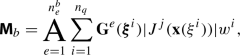(22)

where $$n^b_e$$ is the number of boundary elements, and

\begin{aligned} {\mathbf {G}}^e({\xi }) {=} \left[ \begin{array}{cccc} N_1 ({\xi }) N_1 ({\xi }) &{} N_1 ({\xi }) N_2 ({\xi }) &{} \cdots &{} N_1 ({\xi }) N_{n_b} ({\xi })\\ N_2 ({\xi }) N_1 ({\xi }) &{} N_2 ({\xi }) N_2 ({\xi }) &{} \cdots &{} N_2 ({\xi }) N_{n_b} ({\xi })\\ \vdots &{} \vdots &{} \ddots &{} \vdots \\ N_{n_b} ({\xi }) N_1 ({\xi }) &{} N_{n_b} ({\xi }) N_2 ({\xi }) &{} \cdots &{} N_{n_b} ({\xi }) N_{n_b} ({\xi })\\ \end{array} \right] .\nonumber \\ \end{aligned}
(23)

The right hand side vector for applying the boundary conditions is thus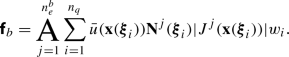(24)

Then the discrete system of equations arising from (21) is

\begin{aligned} {{{\mathbf {\mathsf{{M}}}}}}_b {{{\mathbf {\mathsf{{u}}}}}} = {{{\mathbf {\mathsf{{f}}}}}}_b. \end{aligned}
(25)

We note that the elements for applying boundary conditions are the discretisation of the surface boundary which are one dimensional cubic B-spline curves and only one-dimensional Gauss quadrature rule is used for integration. However, one uses the global degrees of freedom indices to assemble $${{{\mathbf {\mathsf{{M}}}}}}_b$$ and $${{{\mathbf {\mathsf{{f}}}}}}_b$$, so that they have the same size as the system matrix and global right-hand side vector, respectively. Assume the system of equations is expressed as $${{{\mathbf {\mathsf{{K}}}}}} {{{\mathbf {\mathsf{{u}}}}}} = {{{\mathbf {\mathsf{{f}}}}}}$$, where $${{{\mathbf {\mathsf{{K}}}}}}$$ is the system matrix, $${{{\mathbf {\mathsf{{u}}}}}}$$ is the global coefficients vector to be solved for, and $${{{\mathbf {\mathsf{{f}}}}}}$$ is global right-hand side vector. Then, we scale $${{{\mathbf {\mathsf{{M}}}}}}_b$$ and $${{{\mathbf {\mathsf{{f}}}}}}_b$$ using a penalty factor $$\beta$$ and combine them with the systems of equations as

\begin{aligned}{}[{{{\mathbf {\mathsf{{K}}}}}} + \beta {{{\mathbf {\mathsf{{M}}}}}}_b] {{{\mathbf {\mathsf{{u}}}}}} = {{{\mathbf {\mathsf{{f}}}}}} + \beta {{{\mathbf {\mathsf{{f}}}}}}_b. \end{aligned}
(26)

The Dirichlet boundary condition (20) is here weakly applied to the system of equations. A relatively large penalty factor $$\beta = 10^8$$ is selected for all numerical examples. It is sufficiently large to ensure good satisfaction of the constraint but not too large so as to significantly impact the conditioning of the system.

## Laplace–Beltrami problem

The governing partial differential equation which we want to solve to illustrate fundamental features of subdivision surfaces is given by

\begin{aligned} -{\varDelta }_{{\varGamma }} u = f \quad \text {on} \quad {\varGamma }, \end{aligned}
(27)

where $${\varGamma }$$ is a two dimensional manifold (with outward unit normal vector $${\mathbf {n}}$$) in three dimensional space $${\mathbb {R}}^3$$ and $${\varDelta }_{{\varGamma }}(\bullet )$$ is the Laplace–Beltrami operator (also called surface Laplacian operator). The Dirichlet boundary condition is expressed in (20). We will use a manufactured solution to compute against the approximate solution. The Laplace–Beltrami operator is defined by

\begin{aligned} {\varDelta }_{{\varGamma }} (\bullet ) = \nabla _{{\varGamma }}\cdot \nabla _{{\varGamma }}(\bullet ), \end{aligned}
(28)

where $$\nabla _{{\varGamma }}(\bullet )$$ is the surface gradient operator defined by

\begin{aligned} \nabla _{{\varGamma }}(\bullet ) = [{\mathbf {I}} - {\mathbf {n}} \otimes {\mathbf {n}}] \cdot \nabla (\bullet ). \end{aligned}
(29)

Hence the surface gradient of a scalar function v can be calculated as the spatial gradient subtracted by its normal part as

\begin{aligned} \nabla _{{\varGamma }} v = \nabla v - {\mathbf {n}}[{\mathbf {n}} \cdot \nabla v], \end{aligned}
(30)

where $$\nabla (\bullet )$$ is the spatial gradient operator. Hence the surface Laplacian of v is given by

\begin{aligned} {\varDelta }_{{\varGamma }} v= & {} {\varDelta }v - {\mathbf {n}}\cdot [\nabla ^2 v \cdot {\mathbf {n}}] - [{\mathbf {n}} \cdot \nabla v] \nonumber \\&\left[ \nabla \cdot {\mathbf {n}} - {\mathbf {n}} \cdot [\nabla {\mathbf {n}} \cdot {\mathbf {n}}]\right] , \end{aligned}
(31)

where $$\nabla ^2 v$$ is the Hessian matrix of v, and $$\nabla {\mathbf {n}}$$ is the gradient of the normal vector, which is arranged in a matrix as

\begin{aligned} \nabla {\mathbf {n}} = \left[ \begin{array}{ccc} \frac{\partial n_1}{\partial x_1} &{}\frac{\partial n_2}{\partial x_1} &{} \frac{\partial n_3}{\partial x_1}\\ \frac{\partial n_1}{\partial x_2}&{} \frac{\partial n_2}{\partial x_2}&{} \frac{\partial n_3}{\partial x_2}\\ \frac{\partial n_1}{\partial x_3}&{}\frac{\partial n_2}{\partial x_3} &{}\frac{\partial n_3}{\partial x_3} \end{array} \right] . \end{aligned}
(32)

We define the total curvature at a surface point $${\mathbf {x}} \in {\varGamma }$$ as the surface divergence of the normal, that is $$c({\mathbf {x}}) := \nabla _{\varGamma }\cdot {\mathbf {n}}$$. For a given manufactured solution $$u^m$$, the right hand side of Eq. (27) can thus be computed as

\begin{aligned} f({\mathbf {x}})= & {} - {\varDelta }u^m({\mathbf {x}}) + {\mathbf {n}}({\mathbf {x}})\cdot [\nabla ^2 u^m({\mathbf {x}})\cdot \,{\mathbf {n}}({\mathbf {x}})] \nonumber \\&+\, c({\mathbf {x}}) [{\mathbf {n}}({\mathbf {x}}) \cdot \nabla u^m({\mathbf {x}})], \quad {\mathbf {x}} \in {\varGamma }. \end{aligned}
(33)

## Galerkin formulation

The weak formulation of problem (27) is

\begin{aligned} \int _{{\varGamma }} \nabla _{{\varGamma }} u \cdot \nabla _{{\varGamma }} v \, \mathrm {d} {\varGamma }= \int _{{\varGamma }} f v \,\mathrm {d} {\varGamma }, \quad \forall v \in H^1_0({\varGamma }), \end{aligned}
(34)

where v is an admissible test function. The weak formulation is partitioned into $$n_e$$ number of elements, as

\begin{aligned} \sum _{k= 1}^{n_{e}}\int _{{\varGamma }_{e}} \nabla _{{\varGamma }} u \cdot \nabla _{{\varGamma }} v \,\mathrm {d} {\varGamma }= \sum _{k= 1}^{n_{e}}\int _{{\varGamma }_{e}} f v \,\mathrm {d} {\varGamma }. \end{aligned}
(35)

Discretising v, $$\nabla u$$ and $$\nabla v$$ using the Catmull–Clark basis functions $${{\mathbf {N}}}$$ given in Eq. (7) produces

\begin{aligned} v&= \sum _{A = 1}^{n_b} N_{A} v_{A}, \nonumber \\ \nabla _{{\varGamma }} u&= \sum _{A = 1}^{n_b} \nabla _{{\varGamma }} N_A u_A = \sum _{A = 1}^{n_b} \frac{\partial N_A}{\partial \varvec{\xi }}\cdot {\mathbf {J}}^{-1} u_{A}, \nonumber \\ \nabla _{{\varGamma }} v&= \sum _{A = 1}^{n_b} \nabla _{{\varGamma }} N_A v_A = \sum _{A = 1}^{n_b} \frac{\partial N_A}{\partial \varvec{\xi }}\cdot {\mathbf {J}}^{-1} v_{A}, \end{aligned}
(36)

where $${\mathbf {J}}$$ is the surface Jacobian for the manifold, given in a matrix form as

\begin{aligned} {\mathbf {J}} = \frac{\partial {\mathbf {x}}}{\partial \varvec{\xi }} = \left[ \begin{array}{cc} \frac{\partial x_1}{\partial \xi } &{} \frac{\partial x_1}{\partial \eta } \\ \frac{\partial x_2}{\partial \xi } &{} \frac{\partial x_2}{\partial \eta } \\ \frac{\partial x_3}{\partial \xi } &{} \frac{\partial x_3}{\partial \eta } \end{array} \right] . \end{aligned}
(37)

For details on the computation of $${\mathbf {J}}^{-1}$$ see  and for a discussion of superficial tensors such as $${\mathbf {J}}$$ in the context of Laplace–Beltrami equation, see . If the element contains an extraordinary vertex, the shape functions $$N_A$$ are replaced by $${\hat{N}}_A$$ in Eq. (12). The surface gradient of the shape functions is computed as

\begin{aligned} \nabla _{\varGamma }{\hat{N}}_A = \frac{\partial {\hat{N}}_A}{\partial \bar{\varvec{\xi }}} \cdot \frac{\partial \bar{\varvec{\xi }}}{\partial \varvec{\xi }}\cdot {\mathbf {J}}^{-1} \end{aligned}
(38)

and

\begin{aligned} {\mathbf {J}} =\frac{\partial {\mathbf {x}}}{\partial \bar{\varvec{\xi }}} \cdot \frac{\partial \bar{\varvec{\xi }}}{\partial \varvec{\xi }} = \sum _{A=0}^{2\kappa +7} \frac{\partial {\hat{N}}_A}{\partial \bar{\varvec{\xi }}} \, {\mathbf {P}}_{A} \cdot \frac{\partial \bar{\varvec{\xi }}}{\partial \varvec{\xi }}. \end{aligned}
(39)

Integrating the discrete problem using Gauss quadrature, the system of Eq. 34 becomes(40)

where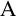is the assembly operator and

\begin{aligned} {\mathbf {D}}^e(\varvec{\xi }) = \left[ \begin{array}{cccc} \nabla _{\varGamma }N_1 (\varvec{\xi }) \cdot \nabla _{\varGamma }N_1 (\varvec{\xi }) &{} \nabla _{\varGamma }N_1 (\varvec{\xi }) \cdot \nabla _{\varGamma }N_2 (\varvec{\xi }) &{} \cdots &{} \nabla _{\varGamma }N_1 (\varvec{\xi }) \cdot \nabla _{\varGamma }N_{n_b} (\varvec{\xi })\\ \nabla _{\varGamma }N_2 (\varvec{\xi }) \cdot \nabla _{\varGamma }N_1 (\varvec{\xi }) &{} \nabla _{\varGamma }N_2 (\varvec{\xi }) \cdot \nabla _{\varGamma }N_2 (\varvec{\xi }) &{} \cdots &{} \nabla _{\varGamma }N_2 (\varvec{\xi }) \cdot \nabla _{\varGamma }N_{n_b} (\varvec{\xi })\\ \vdots &{} \vdots &{} \ddots &{} \vdots \\ \nabla _{\varGamma }N_{n_b} (\varvec{\xi }) \cdot \nabla _{\varGamma }N_1 (\varvec{\xi }) &{} \nabla _{\varGamma }N_{n_b} (\varvec{\xi }) \cdot \nabla _{\varGamma }N_2 (\varvec{\xi }) &{} \cdots &{} \nabla _{\varGamma }N_{n_b} (\varvec{\xi }) \cdot \nabla _{\varGamma }N_{n_b} (\varvec{\xi })\\ \end{array} \right] . \end{aligned}
(41)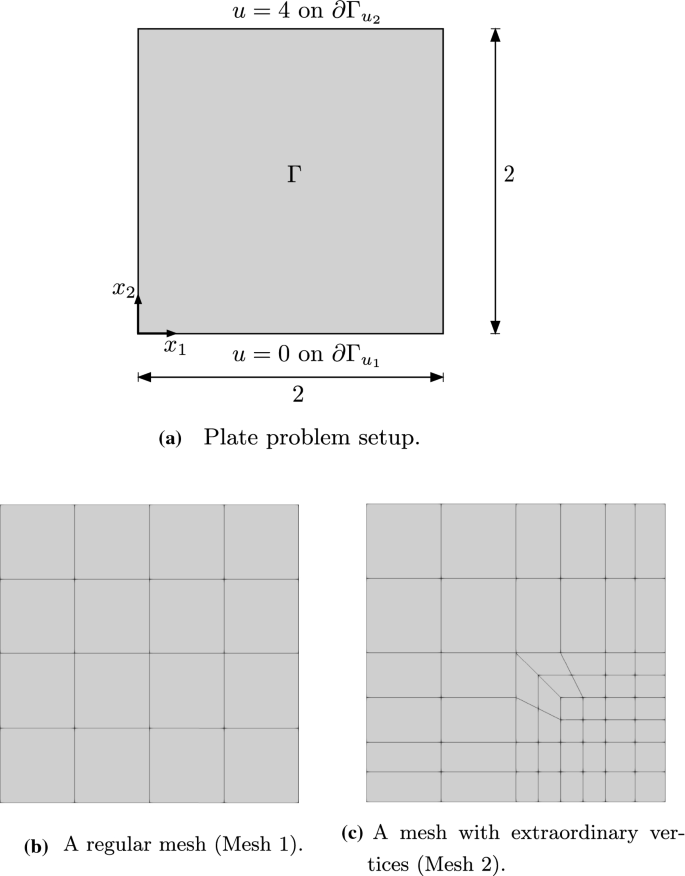Fig. 6

$$n_q$$ is the number of quadrature points in each element, $$w_i$$ is the weight for $$i^\text {th}$$ quadrature point, $$n_e$$ is the number of elements and $$n_b$$ is the number of basis functions of the element. The basis functions $${\textit{\textbf{N}}}^e$$ are replaced by $$\hat{{\textit{\textbf{N}}}}^e$$ if the element e contains an extraordinary vertex. In this case, the basis functions are not differentiable and their derivatives approach positive infinity when points are close to the extraordinary vertex (see Remark 1). Thus $$|{\mathbf {J}}|$$ approaches positive infinity at extraordinary vertices. Errors result if quadrature is adopted to integrate the contributions from element containing extraordinary vertices.

The discrete system of equations to solve is thus given by

\begin{aligned} {{{\mathbf {\mathsf{{K}}}}}} {{{\mathbf {\mathsf{{u}}}}}} = {{{\mathbf {\mathsf{{f}}}}}}. \end{aligned}
(42)

## Numerical results

A ‘patch test’  on a two-dimensional plate is first presented to assess the consistency and stability of the proposed formulation in a simplified setting. Then, the Laplace–Beltrami equation is solved on both cylindrical and hemispherical surfaces. Convergence studies are conducted. The influence of extraordinary vertices is also investigated. All numerical results are computed using an open source finite element library: deal.II [2, 8].

### ‘Patch test’

The ‘patch test’ is performed on a two dimensional flat plate where the Laplace–Beltrami operator reduces to the Laplace operator. The problem proposed in Sect. 4 reduces to the Poisson problem expressed given by

\begin{aligned} -{\varDelta }u = f \quad \text {on} \quad {\varGamma }\in {\mathbb {R}}^2. \end{aligned}
(43)

This partial differential equation is solved on the square plate shown in Fig. 6a with the essential boundary conditions

\begin{aligned} \left\{ \begin{aligned} u = 0 \quad \text {for} \quad x_2 = 0 \quad (\partial {\varGamma }_{u_1})\\ u = 4 \quad \text {for} \quad x_2 = 2 \quad (\partial {\varGamma }_{u_2}) \end{aligned} \right. . \end{aligned}
(44)

The essential boundary conditions are imposed using the penalty method. Natural homogeneous boundary conditions are applied on the remaining two edges of the plate. Four different manufactured functions for f are used. The functions, analytical solutions for u and their gradients $$\frac{\partial u}{\partial x_2}$$ are given in Table 1. We investigate both a regular and an irregular mesh. The regular mesh is a $$4 \times 4$$ element patch without extraordinary vertices as shown in Fig. 6b. In all of the tests, a geometry error is absent.

For Test 1, the right hand side $$f = 0$$ so that $$\frac{\partial u}{\partial x_2} = 2$$. Solving the equation using the proposed Catmull–Clark subdivision method, the numerical result $$u_h$$ is exactly 2 everywhere as shown in Fig. 7b. Thus passes the consistency test and the eigenvalue of the system matrix are all positive and non-zero after application of the essential boundary conditions. The gradient $$\frac{\partial u}{\partial x_2}$$ for Test 2 and 3 are linear and quadratic respectively. Recall that when interpolating functions in elements with edges on physical boundaries, the basis functions are modified, see Eqs. (3) and (4). In other words, the gradients of the function u are expected to be constant at boundaries. Figure 7a, c and e show the numerical results for these tests. The results are smooth and capture the analytical solutions well. Figure 7d and f compare the numerical results of $$\frac{\partial u}{\partial x_2}$$ to the analytical solution for Tests 2 and 3. The Catmull–Clark subdivision method is also compared to linear and quadratic Lagrangian finite element methods. There is a substantial error in both boundary regions in Test 2 for Catmull–Clark subdivision method. This is because the method imposes the gradient to be constant at both boundaries. The numerical result of the Catmull–Clark subdivision method in Test 3 has a substantial error in the region close to the top boundary ($$x_2=2$$) but captures the gradient in the region close to the bottom boundary ($$x_2=0$$) well because the analytic solution for the gradient in the bottom boundary region is near-constant. These errors at the boundaries will pollute the numerical result in the interior of the domain, which will reduce the convergence rate. The gradients approximated by the linear and quadratic Lagrangian finite elements are piecewise constant and piecewise linear, respectively. The results of the Catmull–Clark subdivision methods for these two tests lies between the linear and quadratic Lagrangian elements. The gradient $$\frac{\partial u}{\partial x_2}$$ in Test 4 is a cosine function which is non-polynomial and it behaves as a constant in both boundary regions shown in Fig. 7h. The Lagrangian elements only possess $$C^0$$ continuity across elements and their gradients hence have jumps between elements. The Catmull–Clark subdivision elements capture the gradients of the given function better as they are $$C^1$$ smooth.

Figure 8 shows the plots of normalised global $$L_2$$ and $$H^1$$ errors against the element size. The normalised global $$L_2$$ error is defined by

\begin{aligned} e_{L_2} = \frac{ \Vert u-u_h \Vert _{L_2}}{ \Vert u \Vert _{L_2}}, \end{aligned}
(45)

where $$\Vert \bullet \Vert _{L_2}$$ is the $$L_2$$ norm defined as $$\Vert \bullet \Vert _{L_2} = \sqrt{\int _{\varGamma }|\bullet |^2 \mathrm {d} {\varGamma }}$$. The normalised global $$H^1$$ error is computed as

\begin{aligned} e_{H^1} = \frac{ \Vert u-u_h \Vert _{H^1}}{ \Vert u \Vert _{H^1}}, \end{aligned}
(46)

where $$\Vert \bullet \Vert _{H^1}$$ is the $$H^1$$ norm defined as $$\Vert \bullet \Vert _{H^1} =\sqrt{\int _{\varGamma }|\bullet |^2 \mathrm {d} {\varGamma }+ \int _{\varGamma }| \nabla (\bullet )|^2 \mathrm {d} {\varGamma }}$$. We set the element size of the coarsest mesh as 1. Then, the normalised element size for the refined meshes are $$\frac{1}{2}, \frac{1}{4}, \ldots$$. The convergence rate of Tests 2 and 3 are sub-optimal at 2.5 ($$L_2$$ error) and 1.5 ($$H^1$$ error). The optimal convergence rate for cubic elements should be $$p+1 = 4$$ ($$L_2$$ error) and $$p =3$$ ($$H^1$$ error), where p is the polynomial degree of the basis functions. The numerical result captures the analytical solution well and the convergence rate for Test 4 is optimal. The same convergence study is now repeated starting from a mesh containing extraordinary vertices as shown in Fig. 6c. Figure 8a and b show the plots of normalised element sizes against the $$L_2$$ and $$H^1$$ errors, respectively. The same convergence rates are obtained for Tests 2 and 3. However, the convergence rate of Test 4 is also reduced to 2.5 ($$L_2$$ error) and 1.5 ($$H^1$$ error). Figure 8c and d show the plots of normalised element sizes against $$L_2$$ and $$H^1$$ errors, respectively, for the mesh with an extraordinary vertex.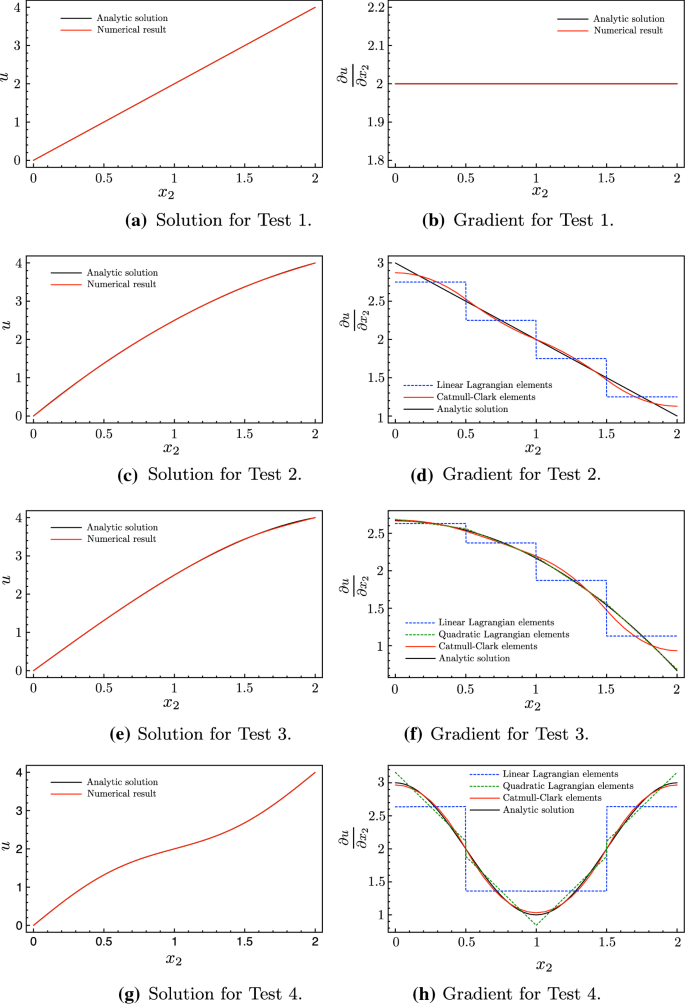Fig. 7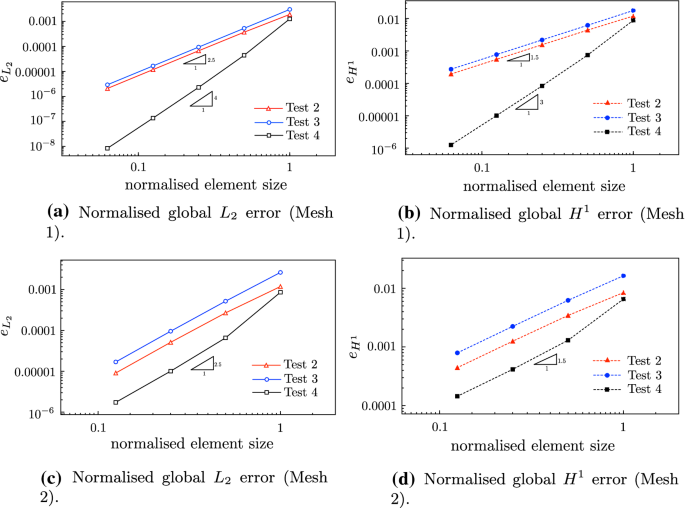Fig. 8

The Catmull–Clark subdivision method can pass the patch test when the function gradient is a constant but has difficulties to capture the gradients in boundary regions when they do not behave like a constant. When the gradient behaves like a constant in the boundary regions, the optimal convergence rate can be obtained. If this is not the case, a reduction of the convergence rate is observed. The presence of the extraordinary vertex in the patch also reduces the convergence rate. It is also important to note that the Catmull–Clark subdivision elements have advantages in describing non-polynomial functions since their basis functions are cubic and $$C^2$$ continuous.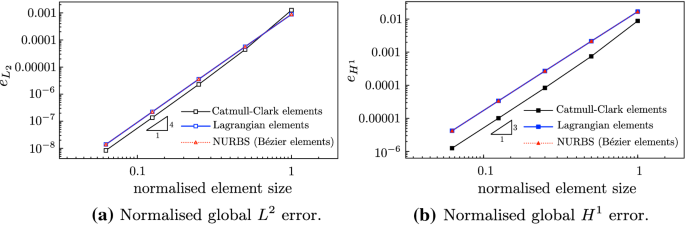Fig. 9

Comparison with NURBS and Lagrangian elements

We now compare the convergence rate associated with Catmull–Clark elements against conventional Lagrangian elements and NURBS. Bézier extraction  is adopted to decompose a NURBS surfaces into $$C_0$$ Bézier elements to provide an element structure for the isogeometric Galerkin method. This is a widely-used method for isogeometric analysis using T-splines . As the Lagrangian and Bézier elements can fully pass the ‘patch test’, they both have no approximation error for Tests 1, 2 and 3. Figure 9 compares their behaviour in approximating non-polynomial solution in Test 4. Mesh 1 is used for all methods. All methods exhibit an optimal convergence rate. Since no geometry error is involved in the ‘patch test’, the Bézier element provides the same performance as the Lagrangian element without the advantages of exact geometry representation. The Catmull–Clark element is slightly more accurate than other two methods for this specific test.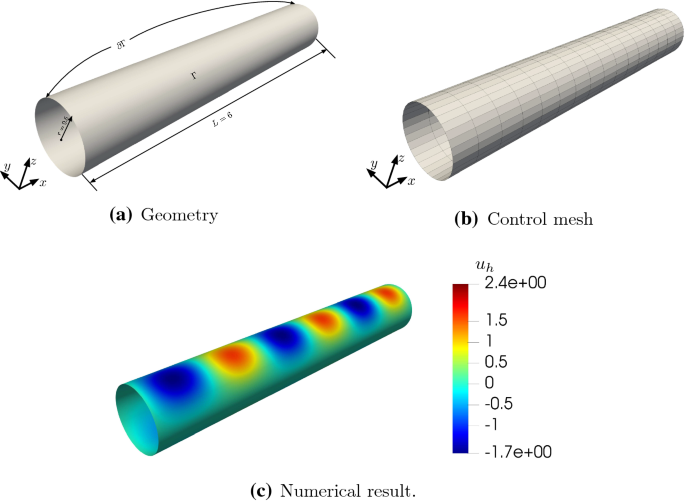Fig. 10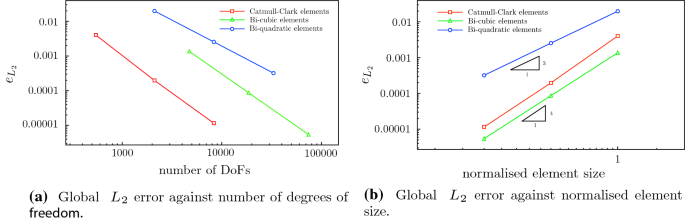Fig. 11

### The Laplace–Beltrami equation

The following sections will solve the Laplace–Beltrami Eq. (27) on different two dimensional Catmull–Clark subdivision manifolds. An analytical solution of function $$u^m$$ is manufactured as

\begin{aligned} u^m ({\mathbf {x}}) = \sin (\pi x_1) \cos (\pi x_2)e^{x_3}, \end{aligned}
(47)

where $${\mathbf {x}}(x_1,x_2,x_3)$$ is a point on the two dimensional manifold in three dimensional space. Applying the Laplacian operator on $$u^m$$ gives

\begin{aligned} {\varDelta }u^u ({\mathbf {x}})= & {} -2\pi ^2 \sin (\pi x_1) \cos (\pi x_2)e^{x_3}\nonumber \\&+ \,\sin (\pi x_1) \cos (\pi x_2)e^{x_3}. \end{aligned}
(48)

Then, the Hessian matrix $$\nabla ^2 u({\mathbf {x}})$$ is computed as

\begin{aligned} \nabla ^2 u^m({\mathbf {x}}) = \left[ \begin{array}{ccc} -\pi ^2 \sin (\pi x_1) \cos (\pi x_2)e^{x_3} &{} -\pi ^2 \cos (\pi x_1) \sin (\pi x_2)e^{x_3} &{} \pi \cos (\pi x_1)\cos (\pi x_2)e^{x_3}\\ -\pi ^2\cos (\pi x_1) \sin (\pi x_2)e^{x_3} &{} -\pi ^2 \sin (\pi x_1) \cos (\pi x_2)e^{x_3} &{} -\pi \sin (\pi x_1) \sin (\pi x_2)e^{x_3}\\ \pi \cos (\pi x_1)\cos (\pi x_2)e^{x_3}&{} -\pi \sin (\pi x_1) \sin (\pi x_2)e^{x_3} &{} \sin (\pi x_1) \cos (\pi x_2)e^{x_3} \end{array} \right] \end{aligned}
(49)

The second term in (33) includes the normal vector $${\mathbf {n}}({\mathbf {x}})$$ and its gradient which can not be computed analytically. In the present work, an $$L^2$$ projection is used to compute the coefficients of normal vectors associated with control points, denoted by $$\hat{{\mathbf {n}}}$$, in order to numerically interpolate the normal vector derivatives at any surface points, thus

\begin{aligned} \frac{\partial {\mathbf {n}}}{\partial {\mathbf {x}}} = \sum _{A = 1}^{n_b} \frac{\partial N_A}{\partial {\mathbf {x}}} \hat{{\mathbf {n}}}_A. \end{aligned}
(50)

#### Cylindrical surface example

The first numerical example considered is a cylindrical surface. The analysis domain of the problem is the cylindrical surface shown in Fig. 10a. Surfaces fitting methods are used to construct the control mesh, see Sect. 3.1. The first level control mesh is shown in Fig. 10b. This has no extraordinary vertices. The Laplace–Beltrami problem on this manifold domain is solved using the Galerkin formulation presented in Sect. 5. Essential boundary conditions are applied on $$\partial {\varGamma }$$. The right-hand side function f is computed using the definition in Eq. (33). Figure 10c shows the numerical result $$u_h$$ which matches the manufactured analytical solution (47) very well.

A convergence study is now conducted for this geometry. The refined control meshes are constructed using the least-squares fitting method described in Sect. 3.1. Figure 11 compares the convergence rates between Catmull–Clark subdivision surfaces with two different order Lagrangian elements. In this example, the shortcoming caused by extraordinary vertices and boundary gradients are not present, and the Catmull–Clark subdivision surfaces have the same convergence rate $$p+1$$ as cubic Lagrangian elements.

#### Hemispherical surface example

The second geometry investigated is a hemispherical surface with radius equal to 1 as shown in Fig. 12a. We use the same strategy to fit the Catmull–Clark subdivision surfaces to the hemispherical surface. The control mesh shown in Fig. 12b is generated to discretise the surface into a number of Catmull–Clark elements. The control mesh has four extraordinary vertices. Figure 12e shows the solution $$u_h$$.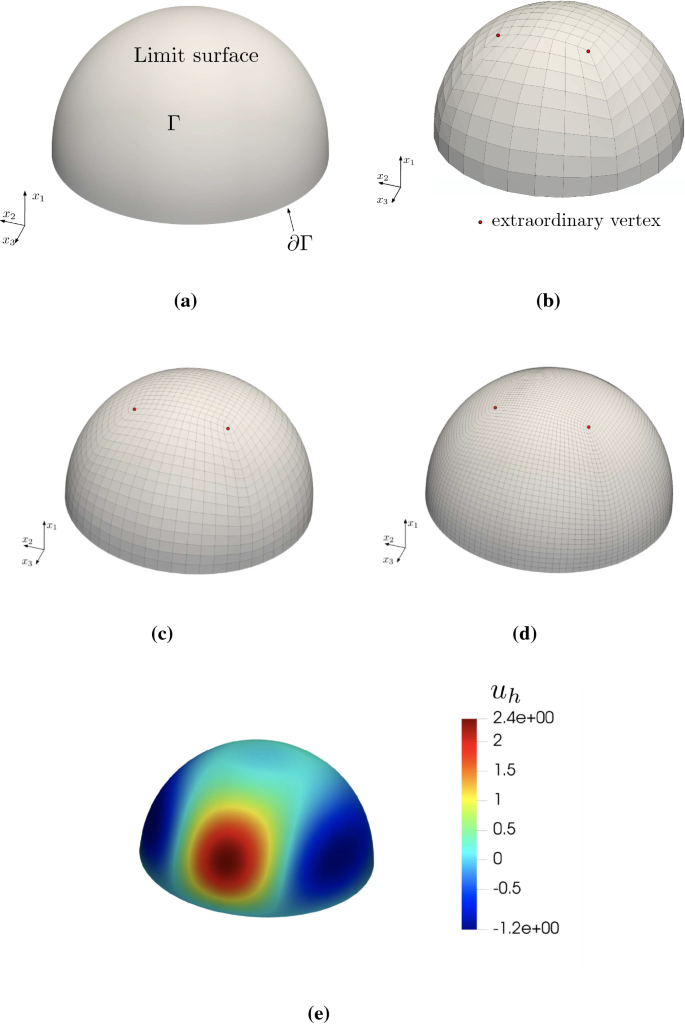Fig. 12Fig. 13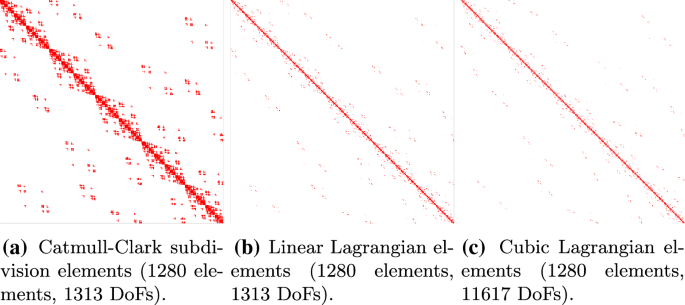Fig. 14

Convergence study with an isogeometric approach

In engineering, designers usually do not know the geometry of the product in advance. The geometry information is purely from the CAD model. Catmull–Clark subdivision surfaces, as a design tool, provide the geometry which is the design of the engineering product. In this case, engineers do not need to approximate the given geometry with Catmull–Clark elements. They can directly adopt the discretisation from the CAD model for analysis. For example, we adopt the control mesh shown in Fig. 12b as the initial control mesh. It can be used to generate a limit surface approximating a hemisphere, as shown in Fig. 12a, with Catmull–Clark subdivision bases. It is important to note the limit surface is not an exact hemisphere since it is evaluated using cubic basis spline functions. However, this surface is the domain of our problem and it will stay exact the same during the entire analysis (isogeometric) and h-refinement with subdivision algorithm will not change the geometry.

The same problem is solved on the subdivision surfaces. A convergence study is done with another two levels of subdivision control mesh as shown in Fig. 12c and d. Note, refinement does not change the number of extraordinary vertices. The two new meshes still have four extraordinary vertices. The two control meshes can be used to evaluate the same limit surface shown in Fig. 12a. The Catmull–Clark subdivision surfaces are compared with quadratic and cubic Lagrangian elements. Generally, Catmull–Clark subdivision elements can achieve higher accuracy per degree of freedom than Lagrangian elements. From the initial to the second level of mesh refinement, the Catmull–Clark subdivision elements have a similar convergence rate to cubic Lagrangian elements. After that, the convergence rate is equivalent to quadratic Lagrangian elements as shown in Fig. 13.

Sparsity patterns

Figure 14a shows the sparsity pattern of the system matrix $${{\mathbf {\mathsf{{K}}}}}$$ for the Catmull–Clark subdivision discretisation. The size of the matrix is the same as the system matrix assembled using a linear Lagrange discretisation. However, because the Catmull–Clark subdivision discretisation uses cubic basis functions with non-local support and there are 16 shape functions in a subdivision element with no extraordinary vertex, the number of non-zero entries in columns and rows is more than the linear Lagrange discretisation (i.e. the sparsity is decreased and the bandwidth increased). Thus, the system matrix of a Catmull–Clark subdivision discretisation has the same size but is denser than the linear Lagrange discretisation shown in 14b. Figure 14c is the sparsity patterns of cubic Lagrange discretisations. p-refinement increase the number of degrees of freedom as well as the number of non-zero entries in rows and columns. Thus there is no significant change in the density of the system matrices. The Catmull–Clark subdivision discretisation has the same number of non-zero entries in each row or column as the cubic Lagrangian discretisation but has a much smaller size.

### Investigation of extraordinary vertices

Quadrature error

The presence of extraordinary vertices leads to difficulties in integration as described in Sect. 3.2. Figure 15a, c and e show the point-wise errors at surface points for three levels of mesh refinement using the standard Gauss quadrature rule. The number of extraordinary vertices remains 4 after refinement. For the analysis using the initial mesh, the error in the regions around extraordinary vertices have similar magnitudes to the other regions. However, after a level of refinement, the error in the other regions is reduced more than the area around the four extraordinary vertices. After the second refinement, the error is concentrated in the areas around the four extraordinary vertices. Figure 15b, d and f plot the point-wise errors on the same mesh analysed with the adaptive quadrature rule shown in Sect. 3.2. The errors around extraordinary vertices are now decreased.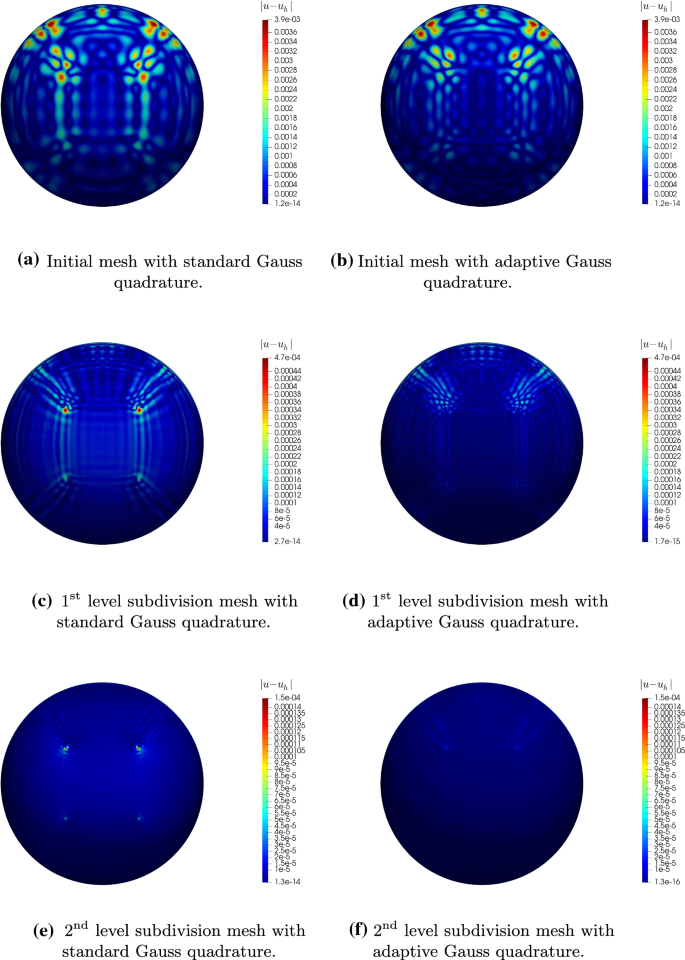Fig. 15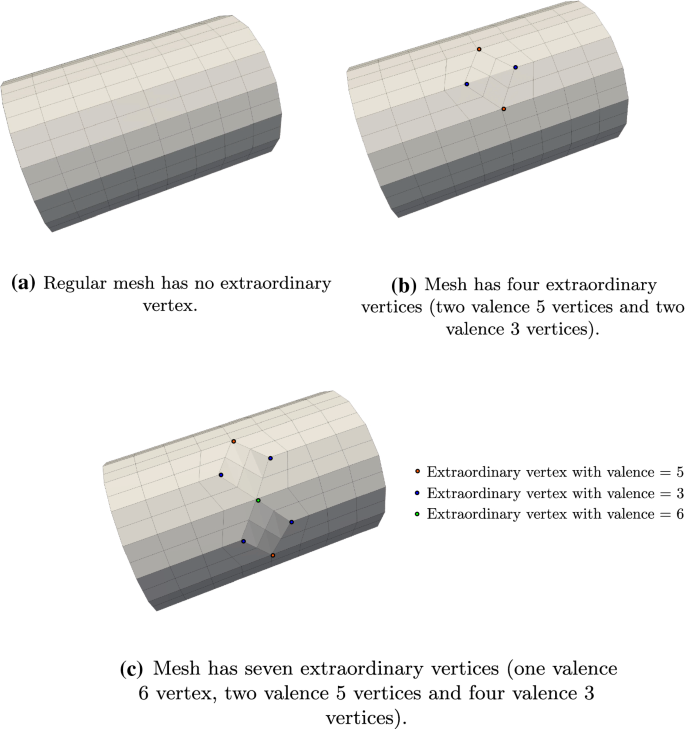Fig. 16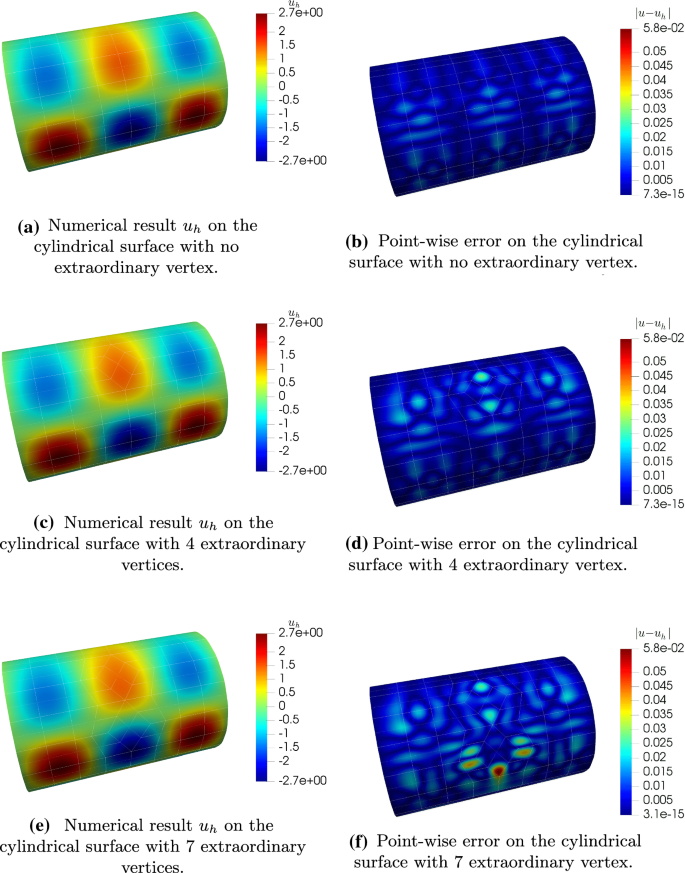Fig. 17

Approximation error

The presence of extraordinary vertices introduces approximation errors. Then we investigate the effect of the number and valence of extraordinary vertices on numerical accuracy. Figure 16a, b and c are three control meshes with different numbers of extraordinary vertices. Figure 16a shows a control mesh without an extraordinary vertex. Figure 16b shows a control mesh with four extraordinary vertices, including two vertices with a valence of 3 and two vertices with a valence of 5. The control mesh in Fig. 16c has seven extraordinary vertices, including four vertices with a valence of 4, two vertices with a valence of 5 and one vertex with a valence of 6. It is important to note the three different control meshes construct different but similar geometries. The Laplace–Beltrami problem is solved using the Galerkin formulation with the same right-hand side function f computed in (33). Both standard and adaptive Gauss quadrature rules are used for all cases. Figure 17a, c and e show the solution of u on the surfaces constructed using the three meshes. Because of the similarity of the geometries and solutions, the three cases are used to investigate the effects of the number of extraordinary vertices in numerical results. The point-wise errors on the three surfaces are shown in Fig. 17b, d and f. Meshes with extraordinary vertices have larger maximum point-wise errors close to the extraordinary vertices, while the mesh without extraordinary vertices has increased uniform point-wise error. Figure 18 shows the convergence rates for the three cases. Meshes without extraordinary vertices can achieve the optimal $$p+1$$ convergence rate and $$p=3$$. In general, the more extraordinary vertices a mesh contains, the more error results. The extraordinary vertices increase the global errors in the results and reduce the convergence rate. Since the global errors also include quadrature errors, the adaptive quadrature rule serves to reduce the quadrature errors. With the adaptive quadrature rule, the convergence rates are improved for the 4 and 7 extraordinary vertices cases but the results still agree with our assumption that increasing the number and valence of extraordinary vertices will produce higher error.

Computational cost

Table 2 compares the computational cost for assembling the system matrix for the standard and adaptive quadrature rules. Because the number of extraordinary vertices remains constant after subdivision, the difference in computational time between the standard and adaptive quadrature schemes diminishes.

### Complex geometry

This final example considers the ability of the Catmull–Clark method to provide high-order discretisations of complex geometry. The model considered is that of a racing car from CAD and imported into Autodesk Maya  for removal of extraneous geometry and the generation of the surface mesh shown in Fig. 19a. Modelling such geometry using NURBS surfaces would require a number of patches to be spliced together. A model based on Catmull–Clark subdivision surface can directly evaluate the smooth limit surface in Fig. 19b using the control mesh containing extraordinary vertices. The minimum bounding box for this model is defined by $$[x_i^{\min }, x_i^{\max }]^3 = [-1.047,1.122]\times [0.097,0.692]\times [-0.460,0.460]$$. The control mesh has 9154 vertices. The physical domain is naturally discretised into a number of elements expressed as

\begin{aligned} {\varGamma }= \bigcup _{e=1}^{n_{e}}{\varGamma }_e, \end{aligned}
(51)

where $$n_e = 9152$$ for this example. Figure 19c indicates the domain where essential (Dirichlet) and natural boundary conditions are applied. The essential boundary $${\varGamma }_d$$ is composed of two parts as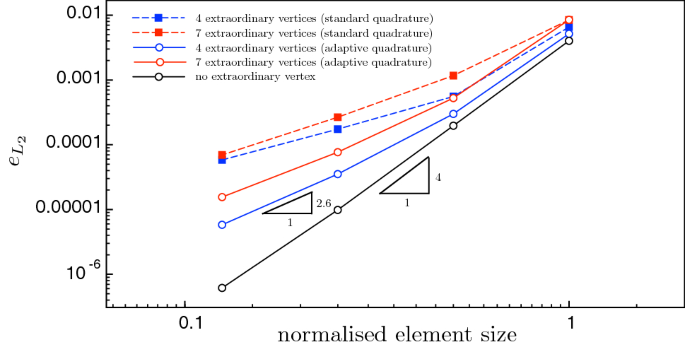Fig. 18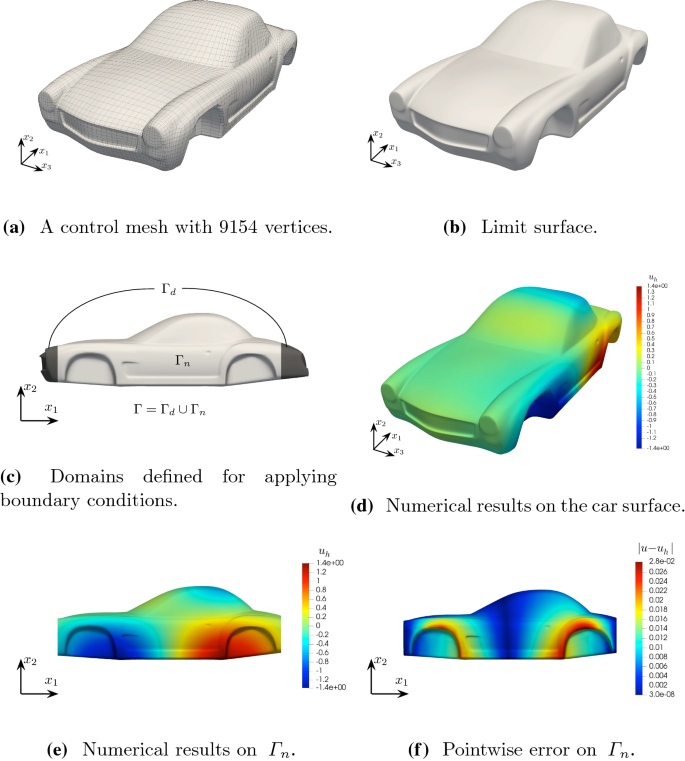Fig. 19
\begin{aligned} {\varGamma }_d = {\varGamma }^1_d + {\varGamma }^1_d, \end{aligned}
(52)

where

\begin{aligned} {\varGamma }^1_d&= \{{\varGamma }_e \,|\, x_2 < -0.9 \quad \forall {\mathbf {x}} \in {\varGamma }_e\}, \end{aligned}
(53)
\begin{aligned} {\varGamma }^2_d&= \{{\varGamma }_e\, | \,x_2 > 0.9 \quad \forall {\mathbf {x}} \in {\varGamma }_e\}. \end{aligned}
(54)

The natural boundary conditions is applied to the rest of the domain $${\varGamma }_n = {\varGamma }\backslash {\varGamma }_d$$. The numerical result matches the analytical solution well as shown in Fig. 19d. Figure 19e shows the results on $${\varGamma }_n$$ and a maximum point-wise error 2.8% is observed in Fig. 19f.

## Conclusions

A thorough study of the isogeometric Galerkin method with Catmull–Clark subdivision surfaces has been presented. The same bases have been used for both geometry and the Galerkin discretisation. The method has been used to solve the Laplace–Beltrami equation on curved two-dimensional manifold embedded in three dimensional space using the Catmull–Clark subdivision surfaces. An approach to fit given geometries using Catmull–Clark subdivision scheme has been outlined. A method to model open boundary geometries without involving ‘ghost’ control vertices, but involving errors in function gradients close to boundary regions, has also been described. The penalty method has been adopted to impose the Dirichlet boundary conditions. The optimal convergence rate of $$p+1$$ has been obtained when using a cylindrical control mesh without extraordinary vertices. A reduction of convergence rates has been observed when the function gradients at the boundaries do not behave like constant, or control meshes contain extraordinary vertices. The adaptive quadrature scheme significantly improves the accuracy. The effect of the number and valence of the extraordinary vertices in convergence rates has been investigated and an adaptive quadrature rule implemented. This successfully improved the convergence rates for the proposed method. The convergence rate of the proposed method is not worse than 2.5 ($$L_2$$ error) and 1.5 ($$H^1$$ error).

In future work, this method will be investigated with problems requiring $$C^1$$ continuity such as the deformations of thin shells.

## References

1. 1.

Antonietti P, Dedner A, Madhavan P, Stangalino S, Stinner B, Verani M (2014) High order discontinuous Galerkin methods on surfaces. arXiv:1402.3428

2. 2.

Arndt D, Bangerth W, Clevenger TC, Davydov D, Fehling M, Garcia-Sanchez D, Harper G, Heister T, Heltai L, Kronbichler M, Kynch RM, Maier M, Pelteret JP, Turcksin B, Wells D (2019) The deal.II library, version 9.1. J Numer Math 27:203–213. https://doi.org/10.1515/jnma-2019-0064

3. 3.

Arnold DN (1982) An interior penalty finite element method with discontinuous elements. SIAM J Numer Anal 19(4):742–760

4. 4.

Autodesk, INC (2019) Maya. https://autodesk.com/maya

5. 5.

Babuška I (1973) The finite element method with Lagrangian multipliers. Numer Math 20(3):179–192

6. 6.

Bandara K, Cirak F (2018) Isogeometric shape optimisation of shell structures using multiresolution subdivision surfaces. Comput Aided Des 95:62–71

7. 7.

Bandara K, Rüberg T, Cirak F (2016) Shape optimisation with multiresolution subdivision surfaces and immersed finite elements. Comput Methods Appl Mech Eng 300:510–539

8. 8.

Bangerth W, Hartmann R, Kanschat G (2007) deal.II—a general purpose object oriented finite element library. ACM Trans Math Softw 33(4):24/1–24/27

9. 9.

Bartezzaghi A, Dedè L, Quarteroni A (2015) Isogeometric analysis of high order partial differential equations on surfaces. Comput Methods Appl Mech Eng 295:446–469

10. 10.

Bazilevs Y, Calo VM, Hughes TJR, Zhang Y (2008) Isogeometric fluid-structure interaction: theory, algorithms, and computations. Comput Mech 43(1):3–37

11. 11.

Bazilevs Y, Calo VM, Cottrell JA, Evans JA, Hughes TJR, Lipton S, Scott MA, Sederberg TW (2010) Isogeometric analysis using T-splines. Comput Methods Appl Mech Eng 199(5–8):229–263

12. 12.

Borden MJ, Scott MA, Evans JA, Hughes TJR (2011) Isogeometric finite element data structures based on Bézier extraction of NURBS. Int J Numer Methods Eng 87(1–5):15–47

13. 13.

Buchegger F, Jüttler B, Mantzaflaris A (2016) Adaptively refined multi-patch B-splines with enhanced smoothness. Appl Math Comput 272:159–172

14. 14.

Catmull E, Clark J (1978) Recursively generated B-spline surfaces on arbitrary topological meshes. Comput Aided Des 10(6):350–355

15. 15.

Chen L, Lu C, Lian H, Liu Z, Zhao W, Li S, Chen H, Bordas SPA (2020) Acoustic topology optimization of sound absorbing materials directly from subdivision surfaces with isogeometric boundary element methods. Comput Methods Appl Mech Eng 362:112806

16. 16.

Cirak F, Ortiz M (2001) Fully $${C}^1$$-conforming subdivision elements for finite deformation thin-shell analysis. Int J Numer Methods Eng 51:813–833

17. 17.

Cirak F, Ortiz M, Schröder P (2000) Subdivision surfaces: a new paradigm for thin-shell finite-element analysis. Int J Numer Methods Eng 47(12):2039–2072

18. 18.

Cottrell JA, Hughes TJR, Bazilevs Y (2009) Isogeometric analysis: toward integration of CAD and FEA. Wiley, Hoboken

19. 19.

Dault D, Li J, Liu B, Zhao R, Tong Y, Shanker B (2015) Subdivision surfaces for electromagnetic integral equations. In: 2015 IEEE international symposium on antennas and propagation USNC/URSI national radio science meeting, pp 151–152

20. 20.

Dedè L, Quarteroni A (2015) Isogeometric analysis for second order partial differential equations on surfaces. Comput Methods Appl Mech Eng 284:807–834

21. 21.

Dedner A, Madhavan P (2016) Adaptive discontinuous Galerkin methods on surfaces. Numer Math 132(2):369–398

22. 22.

Dedner A, Madhavan P, Stinner B (2013) Analysis of the discontinuous Galerkin method for elliptic problems on surfaces. IMA J Numer Anal 33(3):952–973

23. 23.

Deng J, Chen F, Li X, Hu C, Tong W, Yang Z, Feng Y (2008) Polynomial splines over hierarchical T-meshes. Graph Models 70(4):76–86

24. 24.

Dokken T, Lyche T, Pettersen KF (2013) Polynomial splines over locally refined box-partitions. Comput Aided Geom Des 30(3):331–356

25. 25.

Dziuk G (1988) Finite elements for the Beltrami operator on arbitrary surfaces. In: Partial differential equations and calculus of variations. Springer, pp 142–155

26. 26.

Dziuk G, Elliott CM (2007) Surface finite elements for parabolic equations. J Comput Math, pp 385–407

27. 27.

Dziuk G, Elliott CM (2013) Finite element methods for surface PDEs. Acta Numer 22:289–396

28. 28.

Fernández-Méndez S, Huerta A (2004) Imposing essential boundary conditions in mesh-free methods. Comput Methods Appl Mech Eng 193(12–14):1257–1275

29. 29.

Forsey DR, Bartels RH (1988) Hierarchical B-spline refinement. ACM Siggraph Comput Graph 22(4):205–212

30. 30.

Giannelli C, Jüttler B, Speleers H (2012) THB-splines: the truncated basis for hierarchical splines. Comput Aided Geom Des 29(7):485–498

31. 31.

Gurtin ME, Jabbour ME (2002) Interface evolution in three dimensions with curvature-dependent energy and surface diffusion: interface-controlled evolution, phase transitions, epitaxial growth of elastic films. Arch Ration Mech Anal 163(3):171–208

32. 32.

Hansbo A, Hansbo P (2002) An unfitted finite element method, based on Nitsche’s method, for elliptic interface problems. Comput Methods Appl Mech Eng 191(47–48):5537–5552

33. 33.

Hughes TJR, Cottrell JA, Bazilevs Y (2005) Isogeometric analysis: CAD, finite elements, NURBS, exact geometry and mesh refinement. Comput Methods Appl Mech Eng 194(39):4135–4195

34. 34.

Johannessen KA, Kvamsdal T, Dokken T (2014) Isogeometric analysis using LR B-splines. Comput Methods Appl Mech Eng 269:471–514

35. 35.

Jüttler B, Mantzaflaris A, Perl R, Rumpf M (2016) On numerical integration in isogeometric subdivision methods for PDEs on surfaces. Comput Methods Appl Mech Eng 302:131–146

36. 36.

Langer U, Mantzaflaris A, Moore SE, Toulopoulos I (2015) Multipatch discontinuous Galerkin isogeometric analysis. In: Isogeometric analysis and applications 2014. Springer, pp 1–32

37. 37.

Litke N, Levin A, Schröder P (2001) Fitting subdivision surfaces. In: Proceedings of the conference on Visualization’01. IEEE Computer Society, pp 319–324

38. 38.

Liu Z, Majeed M, Cirak F, Simpson RN (2018) Isogeometric FEM–BEM coupled structural-acoustic analysis of shells using subdivision surfaces. Int J Numer Methods Eng 113(9):1507–1530

39. 39.

Moës N, Béchet E, Tourbier M (2006) Imposing Dirichlet boundary conditions in the extended finite element method. Int J Numer Methods Eng 67(12):1641–1669

40. 40.

Neamtu M, Pfluger PR (1994) Degenerate polynomial patches of degree 4 and 5 used for geometrically smooth interpolation in 3. Comput Aided Geom Des 11(4):451–474

41. 41.

Nguyen T, Karčiauskas K, Peters J (2014) A comparative study of several classical, discrete differential and isogeometric methods for solving Poisson’s equation on the disk. Axioms 3(2):280–299

42. 42.

Nguyen-Thanh N, Kiendl J, Nguyen-Xuan H, Wüchner R, Bletzinger KU, Bazilevs Y, Rabczuk T (2011) Rotation free isogeometric thin shell analysis using PHT-splines. Comput Methods Appl Mech Eng 200(47):3410–3424

43. 43.

Nitsche J (1971) Über ein variationsprinzip zur lösung von Dirichlet-problemen bei verwendung von teilräumen, die keinen randbedingungen unterworfen sind. In: Abhandlungen aus dem mathematischen Seminar der Universität Hamburg, Springer 36:9–15

44. 44.

Peters J (1991) Parametrizing singularly to enclose data points by a smooth parametric surface. In: Proceedings of graphics interface, vol 91

45. 45.

Peters J, Reif U (1998) Analysis of algorithms generalizing B-spline subdivision. SIAM J Numer Anal 35(2):728–748

46. 46.

Peterson AF (2005) Mapped vector basis functions for electromagnetic integral equations. Synth Lect Comput Electromagn 1(1):1–124

47. 47.

Reali A (2006) An isogeometric analysis approach for the study of structural vibrations. J Earthq Eng 10(1):1–30

48. 48.

Scott MA, Borden MJ, Verhoosel CV, Sederberg TW, Hughes TJR (2011) Isogeometric finite element data structures based on Bézier extraction of T-splines. Int J Numer Methods Eng 88(2):126–156

49. 49.

Sederberg TW, Zheng J, Bakenov A, Nasri A (2003) T-splines and T-NURCCs. In: ACM transactions on graphics (TOG), vol 22. ACM, pp 477–484

50. 50.

Stam J (1998) Exact evaluation of Catmull–Clark subdivision surfaces at arbitrary parameter values. SIGGRAPH Course Note 98:395–404

51. 51.

Takacs T, Jüttler B (2012) H2 regularity properties of singular parameterizations in isogeometric analysis. Graph Models 74(6):361–372

52. 52.

Vuong A, Giannelli C, Jüttler B, Simeon B (2011) A hierarchical approach to adaptive local refinement in isogeometric analysis. Comput Methods Appl Mech Eng 200(49):3554–3567

53. 53.

Wawrzinek A, Polthier K (2016) Integration of generalized B-spline functions on Catmull–Clark surfaces at singularities. Comput Aided Des 78:60–70

54. 54.

Wei X, Zhang Y, Hughes TJR, Scott MA (2015) Truncated hierarchical Catmull–Clark subdivision with local refinement. Comput Methods Appl Mech Eng 291:1–20

55. 55.

Wei X, Zhang YJ, Hughes TJ, Scott MA (2016) Extended truncated hierarchical Catmull–Clark subdivision. Comput Methods Appl Mech Eng 299:316–336

56. 56.

Xiao X, Sabin M, Cirak F (2019) Interrogation of spline surfaces with application to isogeometric design and analysis of lattice-skin structures. Comput Methods Appl Mech Eng 351:928–950

57. 57.

Zhang Q, Sabin M, Cirak F (2018) Subdivision surfaces with isogeometric analysis adapted refinement weights. Comput Aided Des 102:104–114

58. 58.

Zienkiewicz O, Taylor RL (1997) The finite element patch test revisited a computer test for convergence, validation and error estimates. Comput Methods Appl Mech Eng 149(1–4):223–254

Download references

## Acknowledgements

This work was supported by the UK Engineering and Physical Sciences Research Council grant EP/R008531/1 for the Glasgow Computational Engineering Centre.

## Author information

Authors

### Corresponding author

Correspondence to Zhaowei Liu.

## Additional information

### Publisher's Note

Springer Nature remains neutral with regard to jurisdictional claims in published maps and institutional affiliations.

## Appendix

### Lane–Riesenfeld subdivision algorithm for curves

The Lane–Riesenfeld algorithm successively refines a curve starting from an initial control polygon. After a number of subdivisions, the curve is limited to a B-spline. Figure 20 illustrates a special case of this subdivision algorithm. The control point $${\mathbf {P}}^i_{2j}$$ in the $$i^\text {th}$$ level of refinement is computed from the upper level control points as

\begin{aligned} {\mathbf {P}}^i_{2j} = \frac{1}{2} {\mathbf {P}}^{i-1}_{j} + \frac{1}{2} {\mathbf {P}}^{i-1}_{j+1}. \end{aligned}
(55)

Point $${\mathbf {P}}^i_{2j}$$ is the mid- point of $${\mathbf {P}}^{i-1}_{j}\text{-- }{\mathbf {P}}^{i-1}_{j+1}$$, and is called an ‘edge point’. The control point $${\mathbf {P}}^i_{2j+1}$$ is computed as

\begin{aligned} {\mathbf {P}}^i_{2j+1} = \frac{1}{8} {\mathbf {P}}^{i-1}_{j} + \frac{3}{4} {\mathbf {P}}^{i-1}_{j+1} + \frac{1}{8} {\mathbf {P}}^{i-1}_{j+2}. \end{aligned}
(56)

To compute this point, one needs to connect the mid-points of $${\mathbf {P}}^{i}_{2j}\text{-- }{\mathbf {P}}^{i-1}_{j+1}$$ and $${\mathbf {P}}^{i-1}_{j+1}\text{-- }{\mathbf {P}}^{i}_{2j+2}$$. The point $${\mathbf {P}}^i_{2j+1}$$ is the mid-point of the connecting line. This type of point is called ‘vertex point’. Each ‘vertex point’ is associated with an upper level control point. Figure 21 shows two levels of refinements using the Lane–Riesenfeld algorithm and the limiting result which is a cubic B-spline curve.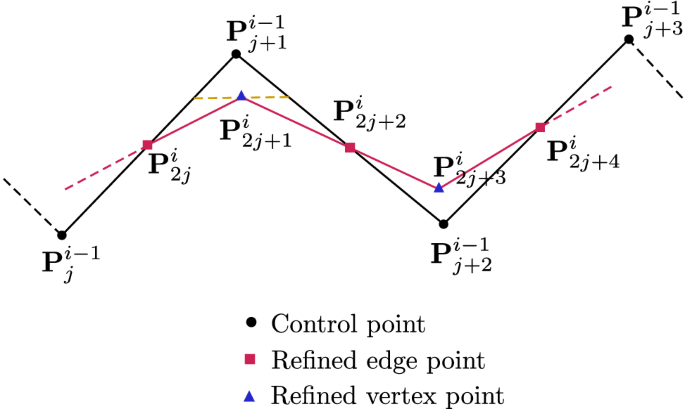Fig. 20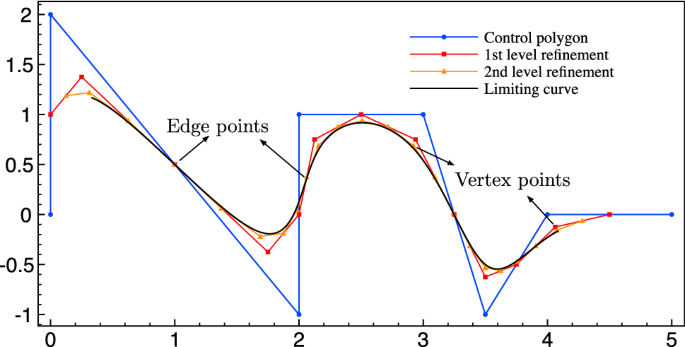Fig. 21

### Catmull–Clark subdivision algorithm for surfaces

The application of the subdivision algorithm to surfaces follows in a similar manner to curves. One face in the original control mesh is split into four new faces. For a closed surface, the numbers of faces and control vertices are doubled. Figure 22 shows an example of generating a new control mesh through the Catmull–Clark algorithm.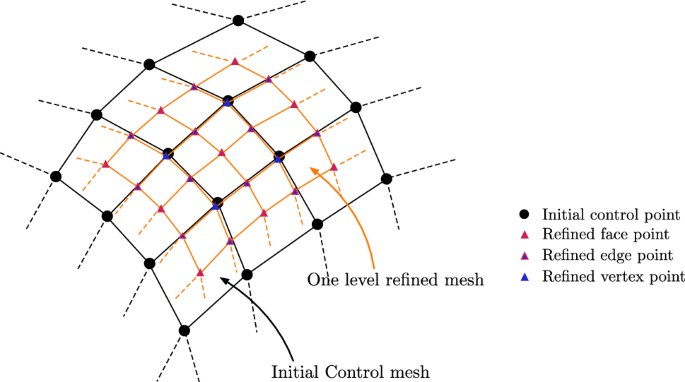Fig. 22

Similar to the one-dimensional curve, the new refined control points can be classified into three types: face points, edge points and vertex points. The face points in the $$i^\text {th}$$ refinement are computed as

\begin{aligned} {\mathbf {P}}^i_{2j,2k} = \frac{1}{4}\left[ {\mathbf {P}}^{i-1}_{j,k}+{\mathbf {P}}^{i-1}_{j,k+1} + {\mathbf {P}}^{i-1}_{j+1,k} + {\mathbf {P}}^{i-1}_{j+1,k+1}\right] , \end{aligned}
(57)

where j and k are indices of control points for orthogonal directions. The face point is the central point of the original face. The edge point is computed as

\begin{aligned} {\mathbf {P}}^i_{2j+1,2k}= & {} \frac{1}{16}\left[ {\mathbf {P}}^{i-1}_{j,k} + 6{\mathbf {P}}^{i-1}_{j,k+1} +{\mathbf {P}}^{i-1}_{j,k+2} +{\mathbf {P}}^{i-1}_{j+1,k} \right. \nonumber \\&\left. +\, 6{\mathbf {P}}^{i-1}_{j+1,k+1} + {\mathbf {P}}^{i-1}_{j+1,k+2}\right] , \end{aligned}
(58)

or likewise

\begin{aligned} {\mathbf {P}}^i_{2j,2k+1}= & {} \frac{1}{16}\left[ {\mathbf {P}}^{i-1}_{j,k} + {\mathbf {P}}^{i-1}_{j,k+1} + 6{\mathbf {P}}^{i-1}_{j+1,k} \right. \nonumber \\&\left. +\,6{\mathbf {P}}^{i-1}_{j+1,k+1} + {\mathbf {P}}^{i-1}_{j+2,k} + {\mathbf {P}}^{i-1}_{j+2,k+1}\right] . \end{aligned}
(59)

The ‘vertex point’ is computed as

\begin{aligned} \begin{aligned} {\mathbf {P}}^i_{2j+1,2k+1}&= \frac{1}{64} \Big [ {\mathbf {P}}^{i-1}_{j,k} + 6{\mathbf {P}}^{i-1}_{j,k+1} +{\mathbf {P}}^{i-1}_{j,k+2} \\&\quad + 6{\mathbf {P}}^{i-1}_{j+1,k} +36{\mathbf {P}}^{i-1}_{j+1,k+1} + 6{\mathbf {P}}^{i-1}_{j+1,k+2}\\&\quad + {\mathbf {P}}^{i-1}_{j+2,k} + 6{\mathbf {P}}^{i-1}_{j+2,k+1} + {\mathbf {P}}^{i-1}_{j+2,k+2}\Big ]. \end{aligned} \end{aligned}
(60)

Equipped with these formulae, the new control points on the $$i\text {th}$$ level of refinement $${\mathscr {P}}^i$$ can be computed as:

\begin{aligned} {\mathscr {P}}^i = {{\mathbf {\mathsf{{S}}}}} {\mathscr {P}}^{i-1}, \end{aligned}
(61)

$${{\mathbf {\mathsf{{S}}}}}$$ is a subdivision operator – a matrix consisting of a set of weights. Each weight is associated with a control point in $${\mathscr {P}}^{i-1}$$. The weight distributions for different types of control points are shown in Fig. 23. The weight distributions for extraordinary point are shown in Fig. 24. After successive levels of refinements, a smooth B-spline surfaces is obtained.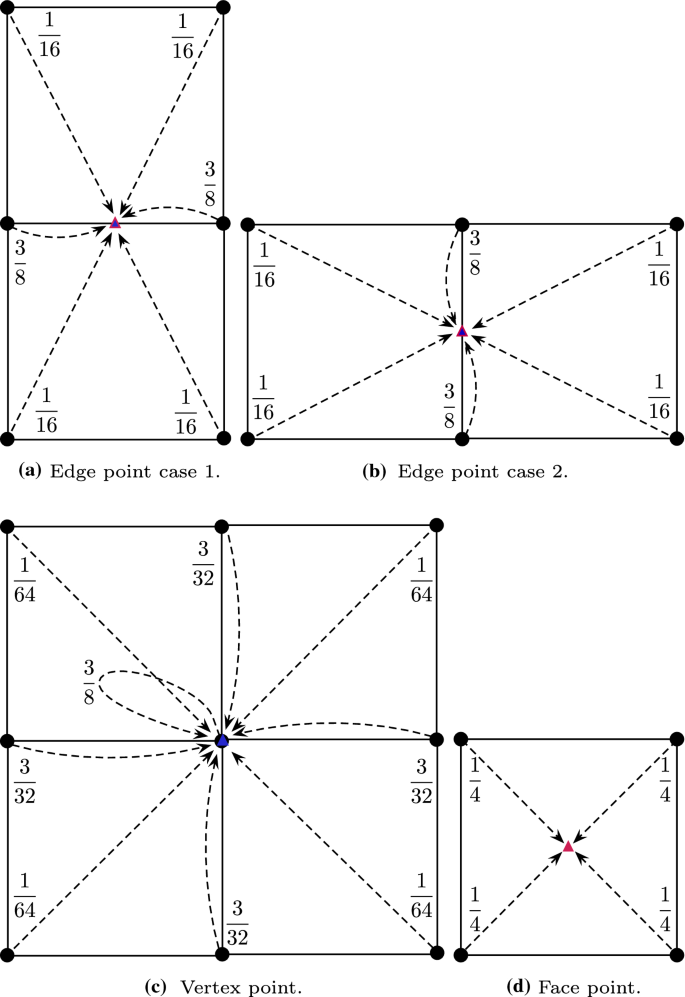Fig. 23

### Computing control point set for sub-elements

We denote the control points of an irregular patch in Fig. 4a as a set $${\mathscr {P}}$$. The initial control points of the patch are expressed as

\begin{aligned} {\mathscr {P}}_0 = \left\{ {\mathbf {P}}^0_{0}, {\mathbf {P}}^0_{1}, \ldots , {\mathbf {P}}^0_{{2\kappa +6}}, {\mathbf {P}}^0_{{2\kappa +7}}\right\} . \end{aligned}
(62)

Through one level of subdivision we generate $$2\kappa + 17$$ new control points ($$\kappa$$ is the valence), as shown in Fig. 4b, denoted by

\begin{aligned} {\mathscr {P}}_1 = \left\{ {\mathbf {P}}^1_{0}, {\mathbf {P}}^1_{1}, \ldots , {\mathbf {P}}^1_{{2\kappa +15}},{\mathbf {P}}^1_{{2\kappa +16}}\right\} . \end{aligned}
(63)

The subdivision step is represented as

\begin{aligned} {\mathscr {P}}_1 = {{\mathbf {\mathsf{{A}}}}}{\mathscr {P}}_0, \end{aligned}
(64)

where $${{{\mathbf {\mathsf{{A}}}}}}$$ is the subdivision operator given by

\begin{aligned} {{\mathbf {\mathsf{{A}}}}} = \left[ \begin{array}{cc} {{\mathbf {\mathsf{{S}}}}} &{} {{\mathbf {\mathsf{{0}}}}}\\ {{\mathbf {\mathsf{{S}}}}}_{11} &{} {{\mathbf {\mathsf{{S}}}}}_{12}\\ {{\mathbf {\mathsf{{S}}}}}_{21} &{} {{\mathbf {\mathsf{{S}}}}}_{22}\\ \end{array} \right] . \end{aligned}
(65)

The terms $${{\mathbf {\mathsf{{S}}}}}$$, $${{\mathbf {\mathsf{{S}}}}}_{11}$$, $${{\mathbf {\mathsf{{S}}}}}_{12}$$, $${{\mathbf {\mathsf{{S}}}}}_{21}$$ and $${{\mathbf {\mathsf{{S}}}}}_{22}$$ are defined in  and $${{\mathbf {\mathsf{{S}}}}}$$ is given in Eq. 61. To evaluate the sub-element $${\varOmega }_1$$, $${\varOmega }_2$$ and $${\varOmega }_3$$ in Fig. 4b, one needs to pick $$2\kappa +8$$ control points out of the new $$2\kappa +17$$ control point patch. A selection operator $${{{\mathbf {\mathsf{{D}}}}}}+\kappa$$ for sub-element $${\varOmega }_k$$ and $$k = 1, 2, 3$$ is used to select the necessary control points from $${\mathscr {P}}_1$$, that is

\begin{aligned} {\mathscr {P}}_{1,k} = {{\mathbf {\mathsf{{D}}}}}_k{\mathscr {P}}_1. \end{aligned}
(66)

Then a surface point can be evaluated with the cubic spline basis functions as

\begin{aligned} {\mathbf {x}}(\varvec{\xi }) = \sum _{A=0}^{15} N_A(\varvec{\xi })\, {\mathbf {P}}^{1,k}_{A}. \end{aligned}
(67)

As shown in Fig. 4c, after successive subdivisions, the non-evaluable element can be limited to a negligible region.

Assume the target point has parametric coordinates $$\varvec{\xi } = (\xi , \eta )$$. One first determines how many subdivisions are required for this point by:

\begin{aligned} n = \left\lfloor {\min \left( -\log _2(\xi ), -\log _2(\eta )\right) +1}\right\rfloor . \end{aligned}
(68)

The sub-element index k is determined as

\begin{aligned} k = \left\{ \begin{aligned}&1 \quad \text {if} \quad \varvec{\xi } \in \left[ \frac{1}{2^n}, \frac{1}{2^{n-1}}\right] \times \left[ 0, \frac{1}{2^n}\right] ,\\&2 \quad \text {if} \quad \varvec{\xi } \in \left[ \frac{1}{2^n}, \frac{1}{2^{n-1}}\right] \times \left[ \frac{1}{2^n}, \frac{1}{2^{n-1}}\right] ,\\&3 \quad \text {if} \quad \varvec{\xi } \in \left[ 0, \frac{1}{2^{n}}\right] \times \left[ \frac{1}{2^n}, \frac{1}{2^{n-1}}\right] .\\ \end{aligned} \right. \end{aligned}
(69)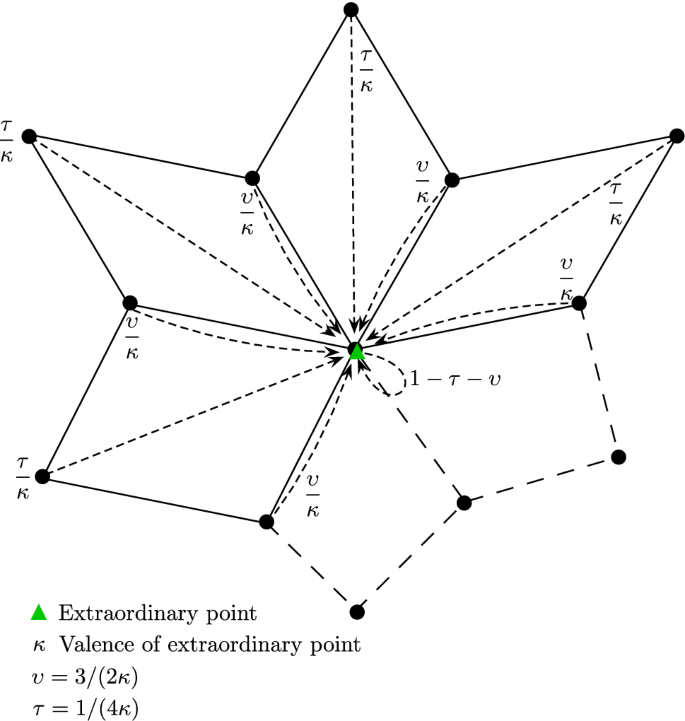Fig. 24

The surface point $${\mathbf {x}}$$ is located in the regular sub-element k after the $$n^\text {th}$$ refinement. The patch for this element is picked with the selection operator $${{\mathbf {\mathsf{{D}}}}}_k$$ as

\begin{aligned} {\mathscr {P}}_{n,k} = {{{\mathbf {\mathsf{{D}}}}}}_k {\mathscr {P}}_n. \end{aligned}
(70)

The enlarged set $${\mathscr {P}}_n$$ contains $$2\kappa + 17$$ control vertices, which is generated from the subdivision of $${{\mathscr {P}}^*}_{n-1}$$ as

\begin{aligned} {\mathscr {P}}_n = {{{\mathbf {\mathsf{{A}}}}}} {{\mathscr {P}}^*}_{n-1}. \end{aligned}
(71)

The set $${{\mathscr {P}}^*}_{n-1}$$ has $$2\kappa + 8$$ control vertices. It is successively refined from the initial set $${\mathscr {P}}_0$$ as

\begin{aligned} {{\mathscr {P}}^*}_{n-1} = \bar{{{{\mathbf {\mathsf{{A}}}}}}}^{n-1} {\mathscr {P}}_0, \end{aligned}
(72)

where $$\bar{{{{\mathbf {\mathsf{{A}}}}}}}$$ is a square matrix operator which subdivides the patch for computing the new patch for the irregular element, and is defined by

\begin{aligned} \bar{{{\mathbf {\mathsf{{A}}}}}} = \left[ \begin{array}{cc} {{\mathbf {\mathsf{{S}}}}} &{} {{\mathbf {\mathsf{{0}}}}}\\ {{\mathbf {\mathsf{{S}}}}}_{11} &{} {{\mathbf {\mathsf{{S}}}}}_{12}\\ \end{array} \right] . \end{aligned}
(73)

## Rights and permissions

Open Access This article is licensed under a Creative Commons Attribution 4.0 International License, which permits use, sharing, adaptation, distribution and reproduction in any medium or format, as long as you give appropriate credit to the original author(s) and the source, provide a link to the Creative Commons licence, and indicate if changes were made. The images or other third party material in this article are included in the article’s Creative Commons licence, unless indicated otherwise in a credit line to the material. If material is not included in the article’s Creative Commons licence and your intended use is not permitted by statutory regulation or exceeds the permitted use, you will need to obtain permission directly from the copyright holder. To view a copy of this licence, visit http://creativecommons.org/licenses/by/4.0/.

Reprints and Permissions